Related Articles
C# Tutorial
• Difficulty Level : Expert
• Last Updated : 20 Jan, 2021

C# is an object-oriented, modern programming language that was created by Microsoft. It runs on the .NET Framework. C# is very close to C/C++ and Java programming languages. It was developed by Anders Hejlsberg and his team within the .NET initiative that approved by the European Computer Manufacturers Association (ECMA) and International Standards Organization (ISO).The first version of C# was released in 2002 and the latest version is 8.0 released in September 2019. Before we start, we must learn about the .NET Framework and Visual Studio.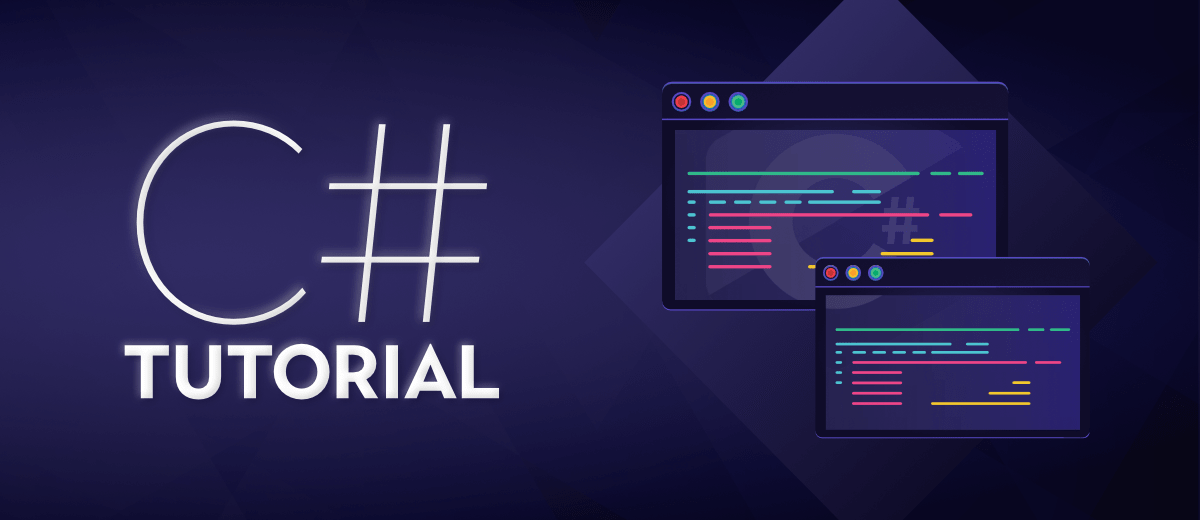Topics:

### .NET Framework & Its Components

.NET is a software framework that is designed and developed by Microsoft. It is a virtual machine for compiling and executing programs written in different languages like C#, VB.Net, etc. It is used to develop Form-based applications, Web-based applications, and Web services. The first version of the .NET framework was 1.0 which came in the year 2002 and the current version is 4.7.1. .NET Framework supports more than 60 programming languages in which C# and VB.Net are main programming languages. Below are the main components of .NET Framework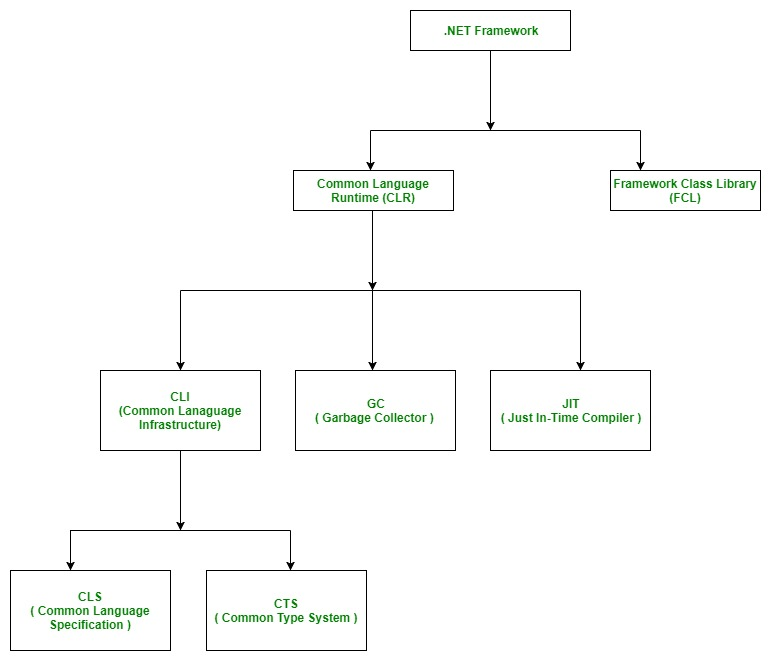1. Common Language Runtime(CLR): CLR is the basic and Virtual Machine component of the `.NET` Framework. It is the run-time environment in the .NET Framework that runs the codes and helps in making the development process easier. It is responsible for managing the execution of `.NET` programs regardless of any .NET programming language. It also helps in the management of code, as code that targets the runtime is known as the Managed Code and code doesn’t target to runtime is known as Unmanaged code. To read more about CLR, you can read the article Common Language Runtime(CLR)

2. Framework Class Library(FCL): It is the collection of reusable, object-oriented class libraries and methods, etc that can be integrated with CLR. Also called the Assemblies. It is just like the header files in C/C++ and packages in the java. Installing `.NET` framework basically is the installation of CLR and FCL into the system. To read more about this, you can read the article .NET Framework Class Library (FCL)

3. Common Intermediate Language: It is a set of instructions that are platform-independent and are generated by the language-specific compiler from the source code. It is also known as the Microsoft Intermediate Language (MSIL). To read more about this, you can read the article Common Intermediate Language(CLI)

4. Garbage Collector(GC): Automatic memory management is made possible by Garbage Collection in .NET Framework. When a class object is created at runtime, certain memory space is allocated to it in the heap memory. However, after all the actions related to the object are completed in the program, the memory space allocated to it is a waste as it cannot be used. In this case, garbage collection is very useful as it automatically releases the memory space after it is no longer required. Garbage collection will always work on Managed Heap and internally it has an Engine which is known as the Optimization Engine. To read more about this, you can refer to the article Garbage Collection in .NET Framework.

5. Just-In-Time(JIT) Compiler: Just-In-Time compiler(JIT) is a part of Common Language Runtime (CLR) in .NET which is responsible for managing the execution of .NET programs regardless of any .NET programming language. A language-specific compiler converts the source code to the intermediate language. This intermediate language is then converted into the machine code by the Just-In-Time (JIT) compiler. This machine code is specific to the computer environment that the JIT compiler runs on. To read more about this, you can read the article What is Just-In-Time(JIT) Compiler in .NET?

6. Managed Code: A code that is written to aimed to get the services of the managed runtime environment execution like CLR(Common Language Runtime) in .NET Framework is known as Managed Code. It always implemented by the managed runtime environment instead of directly executed by the operating system. To read more about this, you can refer to the article Managed code and Unmanaged code in .NET.

7. Unmanaged Code: A code that is directly executed by the operating system is known as Unmanaged code. It always aimed at the processor architecture and depends upon computer architecture. When this code is compiled it always tends to get a specific architecture and always runs on that platform. To read more about this, you can refer to the article Managed code and Unmanaged code in .NET.

### Visual Studio

Visual Studio is an Integrated Development Environment(IDE) developed by Microsoft to develop GUI(Graphical User Interface), console, Web applications, web apps, mobile apps, cloud, and web services, etc. With the help of this IDE, you can create managed code as well as native code. It uses the various platforms of Microsoft software development software like Windows store, Microsoft Silverlight, and Windows API, etc. It is not a language-specific IDE as you can use this to write code in C#, C++, VB(Visual Basic), Python, JavaScript, and many more languages. It provides support for 36 different programming languages. It is available for Windows as well as for macOS. To know more about this, you can refer to the article Getting Familiar With Visual Studio.

### Why C#?

C# has many other reasons for being popular and in demand. Few of the reasons are mentioned below:

1. Easy to Start: C# is a high-level language so it is closer to other popular programming languages like C, C++, and Java and thus becomes easy to learn for anyone.
2. Widely used for developing Desktop and Web Application: C# is widely used for developing web applications and Desktop applications. It is one of the most popular languages that is used in professional desktop. If anyone wants to create Microsoft apps, C# is their first choice.
3. Community:The larger the community the better it is as new tools and software will be developing to make it better. C# has a large community so the developments are done to make it exist in the system and not become extinct.
4. Game Development: C# is widely used in game development and will continue to dominate. C# integrates with Microsoft and thus has a large target audience. The C# features such as Automatic Garbage Collection, interfaces, object-oriented, etc. make C# a popular game developing language.

### Applications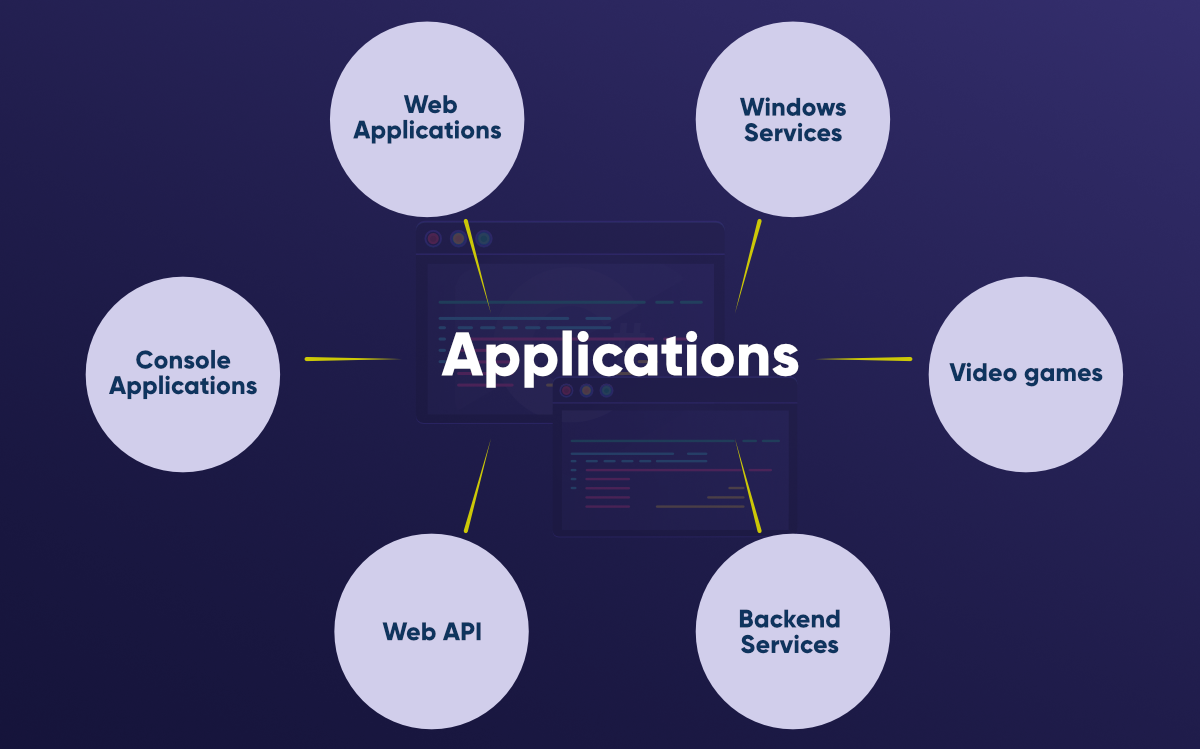### How to Download and Install C# on Windows?

Step 1: Download the Visual Studio Community Version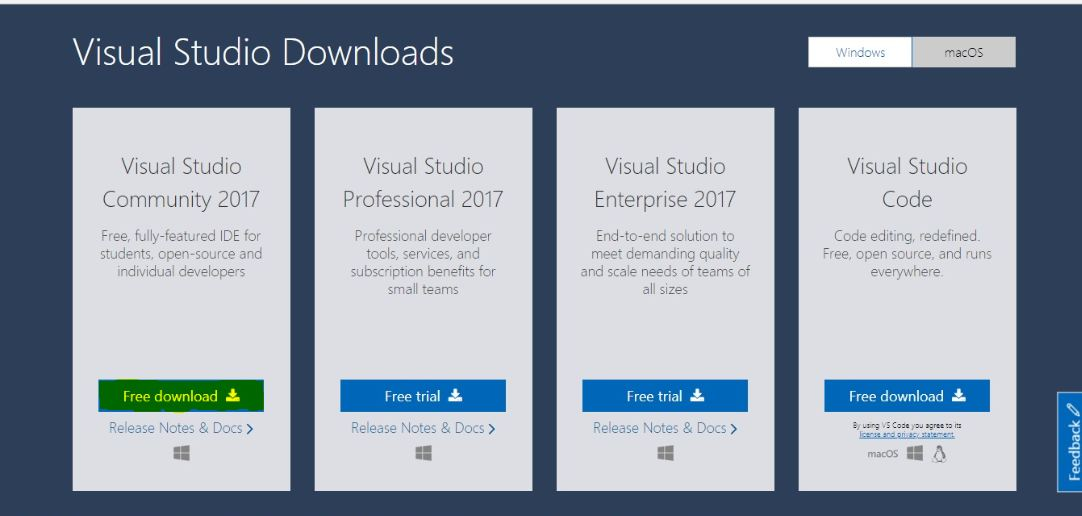Step 2: Run the .exe file and follow the instructions to install Visual Studio Community Version on the system.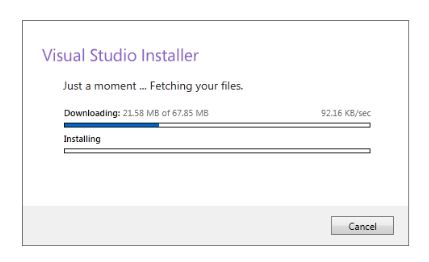Step 3: Select .Net Desktop Development from the options and click to install in bottom right corner as shown below :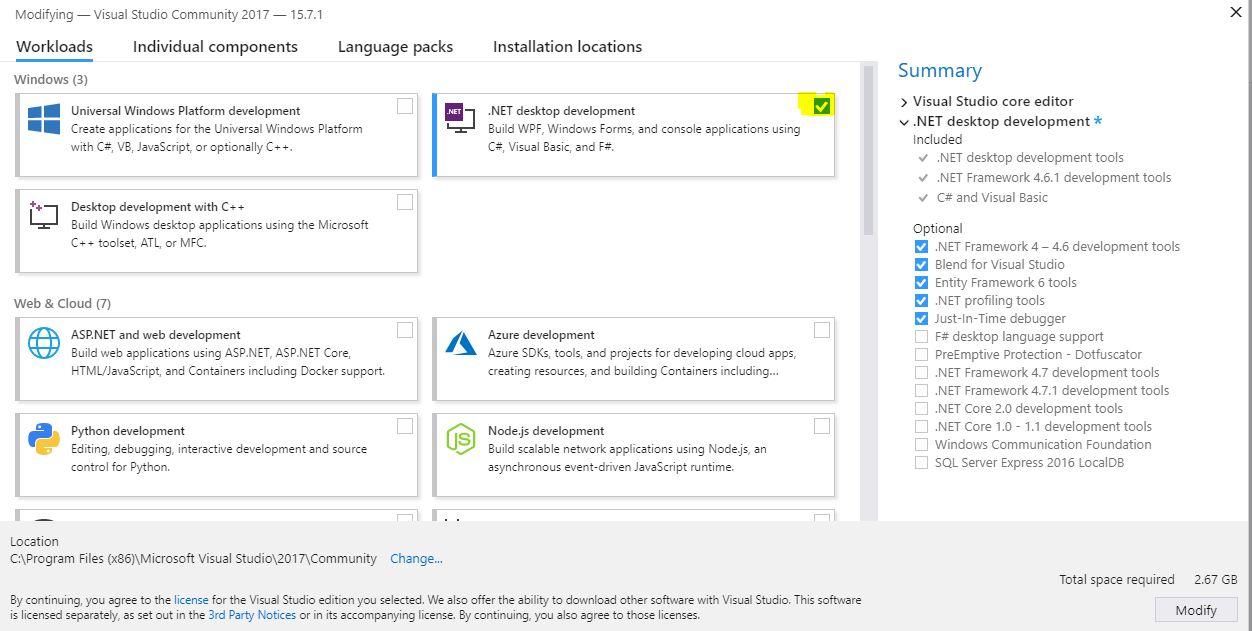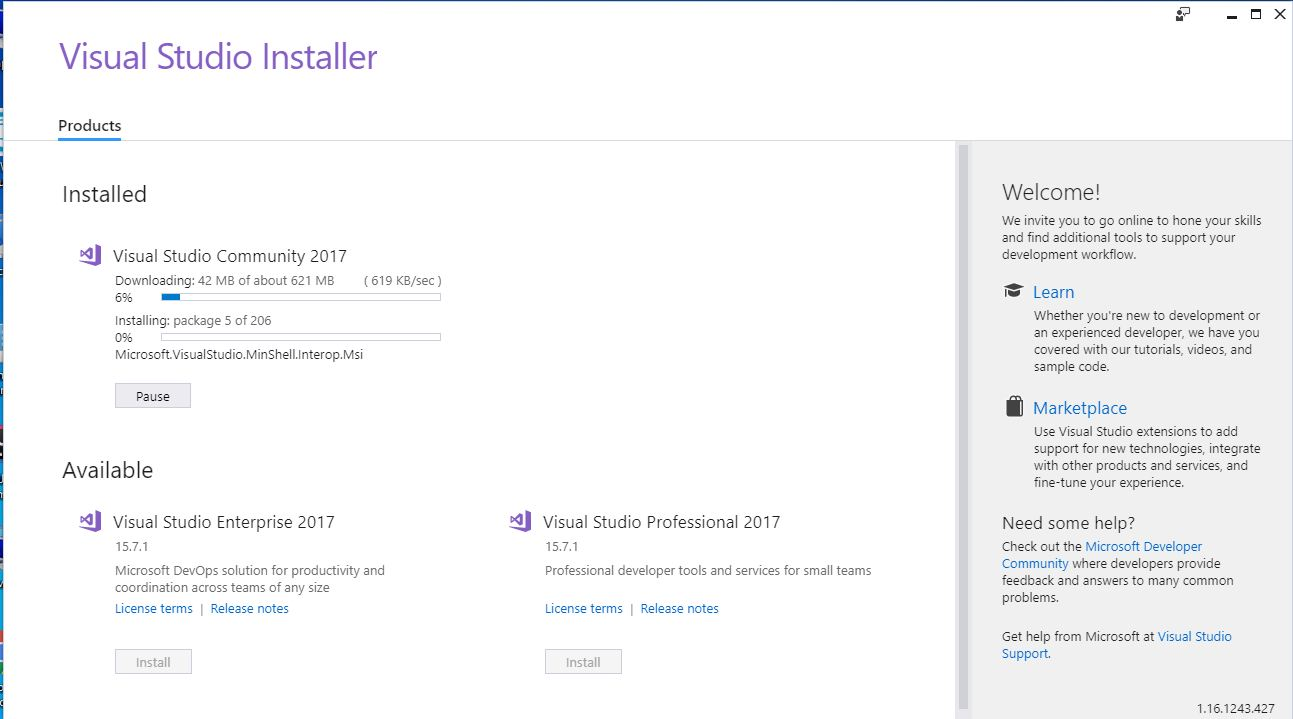Step 4: Open it and it will be prompted to sign in for the first time. The sign-in step is optional so it can be skipped.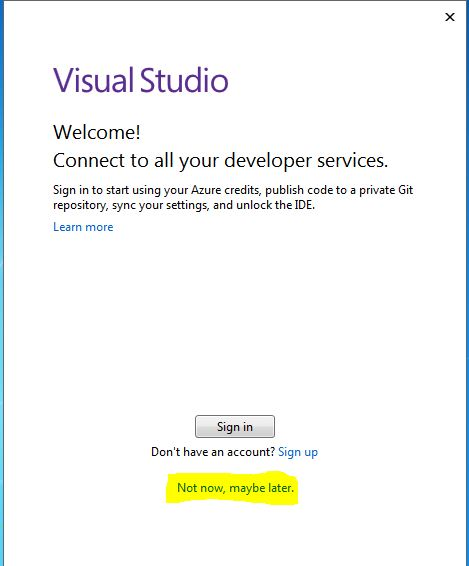Step 5: The dialog box will appear for first time only and ask to choose Development Settings and color theme. Once select required options, click on Start Visual Studio option like as shown below :

Step 6: To create a new console application using C#, Go to File –> New –>Project like as shown below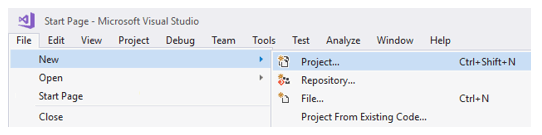Step 7: Choose Console App, write the name of the project and select location path to save project files and then click OK like as shown below.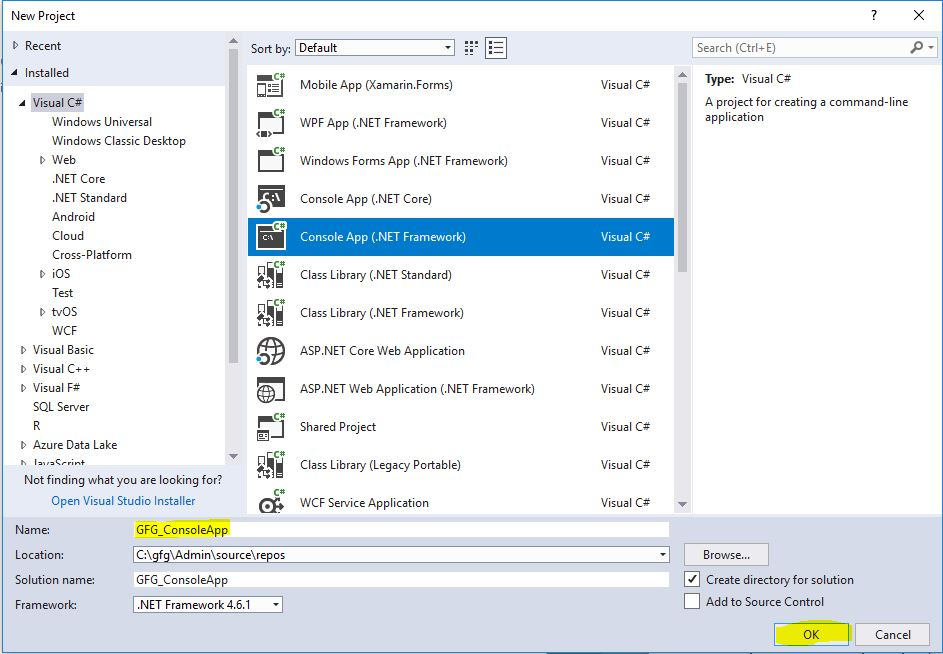Step 8: After clicking OK a predefined template will come and start writing C# code.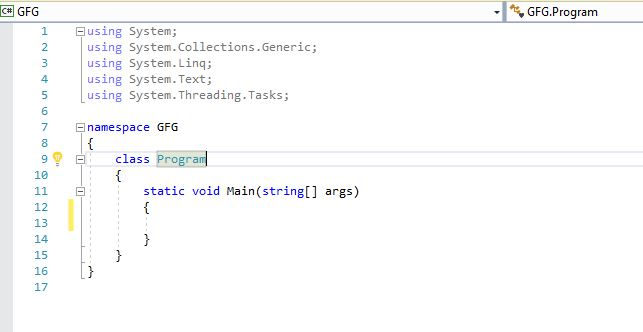### Hello World! Program

Hello World! program is the most basic and first program when you dive into a new programming language. This simply prints the Hello World! on the output screen. In C#, a basic program consists of the following:

• A Namespace Declaration
• Class Declaration & Definition
• Class Members(like variables, methods etc.)
• Main Method
• Statements or Expressions

Example:

 `// C# program to print Hello World!``using` `System;`` ` `// namespace declaration``namespace` `HelloWorldApp {`` ` `// Class declaration``class` `Geeks {`` ` `    ``// Main Method``    ``static` `void` `Main(``string``[] args)``    ``{`` ` `        ``// statement``        ``// printing Hello World!``        ``Console.WriteLine(``"Hello World!"``);`` ` `        ``// To prevents the screen from``        ``// running and closing quickly``        ``Console.ReadKey();``    ``}``}``}`
Output:
```Hello World!
```

Generally, There are 3 ways to compile and execute a C# program as follows:

• To use an online C# compiler: You can use various online IDE. which can be used to run C# programs without installing.
• Using Visual Studio IDE: Microsoft has provided an IDE(Integrated Development Environment) tool named Visual Studio to develop applications using different programming languages such as C#, VB(Visual Basic) etc. To install and use Visual Studio for the commercial purpose it must buy a license from the Microsoft. For learning (non-commercial) purpose, Microsoft provided a free Visual Studio Community Version. To learn how to run a program in Visual Studio you can refer to this.
• Using Command-Line: You can also use command-line options to run a C# program. Below steps demonstrate how to run a C# program on Command line in Windows Operating System:

### Identifiers

In programming languages, identifiers are used for identification purpose. Or in other words, identifiers are the user-defined name of the program components. In C#, an identifier can be a class name, method name, variable name or a label.

Example:

```public class GFG {
static public void Main ()
{
int x;
}
}
```

Here the total number of identifers present in the above example is 3 and the names of these identifiers are:

• GFG: Name of the class
• Main: Method name
• x: Variable name

Rules for defining identifiers in C#:

There are certain valid rules for defining a valid C# identifier. These rules should be followed, otherwise, we will get a compile-time error.

• The only allowed characters for identifiers are all alphanumeric characters([A-Z], [a-z], [0-9]), ‘_‘ (underscore). For example “geek@” is not a valid C# identifier as it contain ‘@’ – special character.
• Identifiers should not start with digits([0-9]). For example “123geeks” is a not a valid in C# identifier.
• Identifiers should not contain white spaces.
• Identifiers are not allowed to use as keyword unless they include @ as a prefix. For example, @as is a valid identifier, but “as” is not because it is a keyword.
• C# identifers allow Unicode Characters.
• C# identifiers are case-sensitive.
• C# identifers cannot contain more than 512 characters.
• Identifiers does not contain two consecutive underscores in its name because such types of identifiers are used for the implementation.

Example:

 `// Simple C# program to illustrate identifiers``using` `System;`` ` `class` `GFG {`` ` `    ``// Main Method``    ``static` `public` `void` `Main()``    ``{`` ` `        ``// variable``        ``int` `a = 10;``        ``int` `b = 39;``        ``int` `c;`` ` `        ``// simple addition``        ``c = a + b;``        ``Console.WriteLine(``"The sum of two number is: {0}"``, c);``    ``}``}`

Output:

`The sum of two number is: 49`

Below table shows the identifers and keywrods present in the above example:

KeywordsIdentifiers
usingGFG
publicMain
statica
voidb
intc

### Keywords

Keywords or Reserved words are the words in a language that are used for some internal process or represent some predefined actions. These words are therefore not allowed to use as variable names or objects. Doing this will result in a compile-time error.

Example:

 `// C# Program to illustrate the keywords``using` `System;`` ` `class` `GFG {`` ` `    ``// Here static, public, void``    ``// are keywords``    ``static` `public` `void` `Main()``    ``{`` ` `        ``// here int is keyword``        ``// a is identifier``        ``int` `a = 10;`` ` `        ``Console.WriteLine(``"The value of a is: {0}"``, a);`` ` `        ``// this is not a valid identifier`` ` `        ``// removing comment will give compile time error``        ``// double int = 10;``    ``}``}`

Output:

`The value of a is: 10`

There are a total of 78 keywords in C# as shown below. To read more this, you can refer to the article Keywords in C#.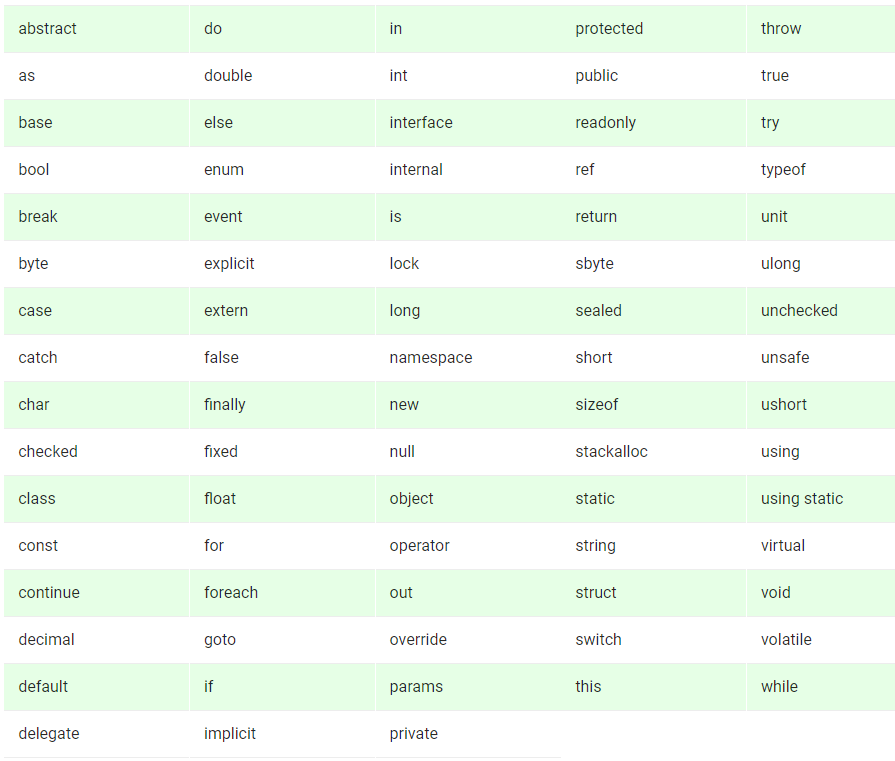## Variables

A Variable is a placeholder of the information which can be changed at runtime. And variables allows to Retrieve and Manipulate the stored information.

Syntax:

```type variable_name = value;
or
type variable_names;
```

Example:

```char var = 'h'; // Declaring and Initializing character variable
int a, b, c; // Declaring variables a, b and c of int type
```

Characteristics of Variables:

• name : It must be a valid identifier. In above example, var is a valid identifier.
• type : It defines the types of information which is to be stored into the variable. In above example char is a type.
• value : It is the actual data which is to be stored in the variable. In above example ‘h’ is the value.

#### Rules for Naming Variables

• Variable names can contain the letters ‘a-z’ or ’A-Z’ or digits 0-9 as well as the character ‘_’.
• The name of the variables cannot be started with a digit.
• The name of the variable cannot be any C# keyword say int, float, null, String, etc.

Examples:

• Valid Variables Names
```int age;

float _studentname;```
• Invalid Variables Names
```int if; // "if" is a keyword

float 12studentname; // Cannot start with digit
```

#### Defining or Declaring a Variable

There are some rules that must be followed while declaring variables :

• specify its type (such as int)
• specify its name (such as age)
• Can provide initial value(such as 17)

Example :

```int geeks;
float interest;
```

#### Initializing Variables

The term initializing means to assign some value to the variable. Basically, the actual use of variables comes under the initialization part. In C# each data type has some default value which is used when there is no explicitly set value for a given variable. Initialization can be done separately or may be with declaration.

Example :

```int y = 7; // Declaring and initializing the variable at same time
int x; // Declaring variable x
x = 5; // initializing x with value 5```

#### Two Ways for Initialization:

1. Compile time initialization
2. Run time initialization

To read about these, you can refer to the article Variables in C#

### Literals

The fixed values are called as Literal. Literal is a value which is used by the variables. Values can be either an integer, float or string etc.

```// Here 100 is a constant/literal.
int x = 100;
```

Literals can be of following types:

• Integer Literals
• Floating-point Literals
• Character Literals
• String Literals
• Null Literals
• Boolean Literals

Example:

 `// C# program to illustrate the use of Literals``using` `System;`` ` `class` `Geeks {`` ` `    ``// Main method``    ``public` `static` `void` `Main(String[] args)``    ``{``        ``// decimal-form literal``        ``int` `a = 101;`` ` `        ``// floating-point literal``        ``double` `b = 101.230;`` ` `        ``Console.WriteLine(a);``        ``Console.WriteLine(b);`` ` `        ``// character literal within single quote``        ``char` `ch = ``'a'``;`` ` `        ``// Unicode representation``        ``char` `c = ``'\u0061'``;`` ` `        ``Console.WriteLine(ch);``        ``Console.WriteLine(c);`` ` `        ``// Escape character literal``        ``Console.WriteLine(``"Hello\n\nGeeks\t!"``);``    ``}``}`

Output:

```101
101.23
a
a
Hello

Geeks    !
```

To know more about literals, you can refer to the article Literals in C#

### Data Types

Data types specify the type of data that a valid C# variable can hold. C# is a strongly typed programming language because in C#, each type of data (such as integer, character, float, and so forth) is predefined as part of the programming language and all constants or variables defined for a given program must be described with one of the data types. In C#, data types are divided into 3 categories as shown below: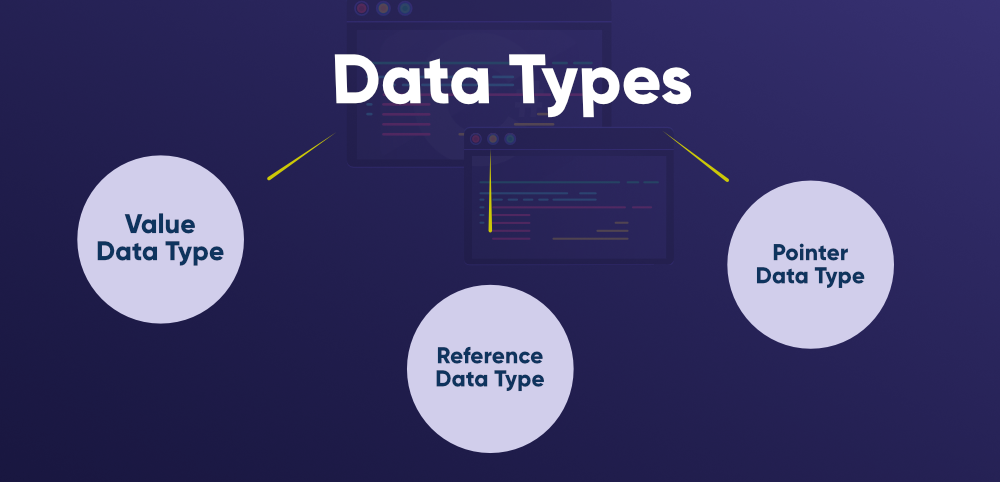1. Value Data Types : In C#, the Value Data Types will directly store the variable value in memory and it will also accept both signed and unsigned literals. The derived class for these data types are System.ValueType. Following are different Value Data Types in C# programming language

• Signed & Unsigned Integral Types
• Floating Point Types
• Decimal Types
• Character Types
• Boolean Types

Example 1:

 `// C# program to demonstrate``// the above data types``using` `System;``namespace` `ValueTypeTest {`` ` `class` `GeeksforGeeks {`` ` `    ``// Main function``    ``static` `void` `Main()``    ``{`` ` `        ``// declaring character``        ``char` `a = ``'G'``;`` ` `        ``// Integer data type is generally``        ``// used for numeric values``        ``int` `i = 89;`` ` `        ``short` `s = 56;`` ` `        ``// this will give error as number``        ``// is larger than short range``        ``// short s1 = 87878787878;`` ` `        ``// long uses Integer values which``        ``// may signed or unsigned``        ``long` `l = 4564;`` ` `        ``// UInt data type is generally``        ``// used for unsigned integer values``        ``uint` `ui = 95;`` ` `        ``ushort` `us = 76;``        ``// this will give error as number is``        ``// larger than short range`` ` `        ``// ulong data type is generally``        ``// used for unsigned integer values``        ``ulong` `ul = 3624573;`` ` `        ``// by default fraction value``        ``// is double in C#``        ``double` `d = 8.358674532;`` ` `        ``// for float use 'f' as suffix``        ``float` `f = 3.7330645f;`` ` `        ``// for float use 'm' as suffix``        ``decimal` `dec = 389.5m;`` ` `        ``Console.WriteLine(``"char: "` `+ a);``        ``Console.WriteLine(``"integer: "` `+ i);``        ``Console.WriteLine(``"short: "` `+ s);``        ``Console.WriteLine(``"long: "` `+ l);``        ``Console.WriteLine(``"float: "` `+ f);``        ``Console.WriteLine(``"double: "` `+ d);``        ``Console.WriteLine(``"decimal: "` `+ dec);``        ``Console.WriteLine(``"Unsinged integer: "` `+ ui);``        ``Console.WriteLine(``"Unsinged short: "` `+ us);``        ``Console.WriteLine(``"Unsinged long: "` `+ ul);``    ``}``}``}`

Output :

```char: G
integer: 89
short: 56
long: 4564
float: 3.733064
double: 8.358674532
decimal: 389.5
Unsinged integer: 95
Unsinged short: 76
Unsinged long: 3624573
```

Example 2:

 `// C# program to demonstrate the``// boolean data type``using` `System;``namespace` `ValueTypeTest {`` ` `class` `GeeksforGeeks {`` ` `    ``// Main function``    ``static` `void` `Main()``    ``{`` ` `        ``// boolean data type``        ``bool` `b = ``true``;``        ``if` `(b == ``true``)``            ``Console.WriteLine(``"Hi Geek"``);``    ``}``}``}`

Output :

```Hi Geek
```

2. Reference Data Types: The Reference Data Types will contain a memory address of variable value because the reference types won’t store the variable value directly in memory. The built-in reference types are string, object.

Example:

 `// C# program to demonstrate``// the Reference data types``using` `System;``namespace` `ValueTypeTest {`` ` `class` `GeeksforGeeks {`` ` `    ``// Main Function``    ``static` `void` `Main()``    ``{`` ` `        ``// declaring string``        ``string` `a = ``"Geeks"``;`` ` `        ``// append in a``        ``a += ``"for"``;``        ``a = a + ``"Geeks"``;``        ``Console.WriteLine(a);`` ` `        ``// declare object obj``        ``object` `obj;``        ``obj = 20;``        ``Console.WriteLine(obj);`` ` `        ``// to show type of object``        ``// using GetType()``        ``Console.WriteLine(obj.GetType());``    ``}``}``}`

Output :

```GeeksforGeeks
20
System.Int32
```

3. Pointer Data Type : The Pointer Data Types will contain a memory address of the variable value. To get the pointer details we have a two symbols ampersand (&) and asterisk (*).

• ampersand (&): It is Known as Address Operator. It is used to determine the address of a variable.
• asterisk (*): It also known as Indirection Operator. It is used to access the value of an address.

Syntax:

```type* identifier;
```

Example :

```int* p1, p;   // Valid syntax
int *p1, *p;   // Invalid
```

Example:

 `// Note: This program will not work on``// online compiler``// Error: Unsafe code requires the `unsafe'``// command line option to be specified``// For its solution:``// Go to your project properties page and``// check under Build the checkbox Allow``// unsafe code.``using` `System;``namespace` `Pointerprogram {`` ` `class` `GFG {`` ` `    ``// Main function``    ``static` `void` `Main()``    ``{``        ``unsafe``        ``{`` ` `            ``// declare variable``            ``int` `n = 10;`` ` `            ``// store variable n address``            ``// location in pointer variable p``            ``int``* p = &n;``            ``Console.WriteLine(``"Value :{0}"``, n);``            ``Console.WriteLine(``"Address :{0}"``, (``int``)p);``        ``}``    ``}``}``}`

To gain in-depth knowledge about data types, you can read the article Data Types in C#

### Operators

Operators are the foundation of any programming language. Thus the functionality of C# language is incomplete without the use of operators. Operators allow us to perform different kinds of operations on operands. In C#, operators Can be categorized based upon their different functionality :

In C#, Operators can also categorized based upon Number of Operands :

• Unary Operator : Operator that takes one operand to perform the operation.
• Binary Operator : Operator that takes two operands to perform the operation.
• Ternary Operator : Operator that takes three operands to perform the operation.

Example:

 `// C# program to demonstrate``// the use of operators``using` `System;`` ` `class` `GFG {`` ` `    ``// Main Function``    ``static` `void` `Main(``string``[] args)``    ``{``        ``int` `result1, result4, result5;``        ``bool` `result2, result3;``        ``int` `x = 10, y = 5;`` ` `        ``// Using Arithmetic operator``        ``result1 = (x + y);``        ``Console.WriteLine(``"Addition Operator: "` `+ result1);`` ` `        ``// Using Relation operator``        ``result2 = (x == y);``        ``Console.WriteLine(``"Equal to Operator: "` `+ result2);`` ` `        ``bool` `a = ``true``, b = ``false``;`` ` `        ``// Using Logical Operator``        ``result3 = a && b;``        ``Console.WriteLine(``"AND Operator: "` `+ result3);`` ` `        ``// Using Bitwise Operator``        ``result4 = x & y;``        ``Console.WriteLine(``"Bitwise AND: "` `+ result4);`` ` `        ``// Using Assignment Operator`` ` `        ``// initalize variable m``        ``// using Simple Assignment``        ``// Operator "="``        ``int` `m = 15;`` ` `        ``// it means m = m + 10``        ``m += 10;``        ``Console.WriteLine(``"Add Assignment Operator: "` `+ m);`` ` `        ``// Using Conditional Operator`` ` `        ``// To find which value is greater``        ``// Using Conditional Operator``        ``result5 = x < y ? x : y;`` ` `        ``// To display the result``        ``Console.WriteLine(``"Result: "` `+ result5);``    ``}``}`

Output:

```Addition Operator: 15
Equal to Operator: False
AND Operator: False
Bitwise AND: 0
Add Assignment Operator: 25
Result: 5
```

To know more about operators, you can read the article Operators in C#

### Enumeration

Enumeration (or enum) is a value data type in C#. It is mainly used to assign the names or string values to integral constants, that make a program easy to read and maintain. For example, the 4 suits in a deck of playing cards may be 4 enumerators named Club, Diamond, Heart, and Spade, belonging to an enumerated type named Suit. Other examples include natural enumerated types (like the planets, days of the week, colors, directions, etc.). The main objective of enum is to define our own data types(Enumerated Data Types). Enumeration is declared using enum keyword directly inside a namespace, class, or structure.

Syntax:

```enum Enum_variable
{
string_1...;
string_2...;
.
.
}
```

In the above syntax, Enum_variable is the name of the enumerator, and string_1 is attached with value 0, string_2 is attached value 1 and so on. Because by default, the first member of an enum has the value 0 and the value of each successive enum member is increased by 1. We can change this default value.

Example: Consider the below code for the enum. Here enum with name month is created and its data members are the name of months like jan, feb, mar, apr, may. Now let’s try to print the default integer values of these enums. An explicit cast is required to convert from enum type to an integral type.

 `// C# program to illustrate the enums``// with their default values``using` `System;``namespace` `ConsoleApplication1 {`` ` `// making an enumerator 'month'``enum` `month {`` ` `    ``// following are the data members``    ``jan,``    ``feb,``    ``mar,``    ``apr,``    ``may`` ` `}`` ` `class` `Program {`` ` `    ``// Main Method``    ``static` `void` `Main(``string``[] args)``    ``{`` ` `        ``// getting the integer values of data members``        ``Console.WriteLine(``"The value of jan in month "``                          ``+ ``"enum is "` `+ (``int``)month.jan);``        ``Console.WriteLine(``"The value of feb in month "``                          ``+ ``"enum is "` `+ (``int``)month.feb);``        ``Console.WriteLine(``"The value of mar in month "``                          ``+ ``"enum is "` `+ (``int``)month.mar);``        ``Console.WriteLine(``"The value of apr in month "``                          ``+ ``"enum is "` `+ (``int``)month.apr);``        ``Console.WriteLine(``"The value of may in month "``                          ``+ ``"enum is "` `+ (``int``)month.may);``    ``}``}``}`
Output:
```The value of jan in month enum is 0
The value of feb in month enum is 1
The value of mar in month enum is 2
The value of apr in month enum is 3
The value of may in month enum is 4
```

To read more about this, you can refer to the article Enum in C#.

### Decision Making Statements

Decision Making in programming is similar to decision making in real life. In programming too, a certain block of code needs to be executed when some condition is fulfilled.
A programming language uses control statements to control the flow of execution of the program based on certain conditions. These are used to cause the flow of execution to advance and branch based on changes to the state of a program. The main conditional statements of C#:

Example 1:

 `// C# program to illustrate if statement``using` `System;`` ` `public` `class` `GFG {`` ` `    ``public` `static` `void` `Main(``string``[] args)``    ``{``        ``string` `name = ``"Geek"``;``        ``if` `(name == ``"Geek"``) {``            ``Console.WriteLine(``"GeeksForGeeks"``);``        ``}``    ``}``}`

Output:

`GeeksForGeeks`

Example 2:

 `// C# program to illustrate``// if-else-if ladder``using` `System;`` ` `class` `GFG {`` ` `    ``public` `static` `void` `Main(String[] args)``    ``{``        ``int` `i = 20;`` ` `        ``if` `(i == 10)``            ``Console.WriteLine(``"i is 10"``);``        ``else` `if` `(i == 15)``            ``Console.WriteLine(``"i is 15"``);``        ``else` `if` `(i == 20)``            ``Console.WriteLine(``"i is 20"``);``        ``else``            ``Console.WriteLine(``"i is not present"``);``    ``}``}`

Output:

`i is 20`

### Switch Statement

In C#, Switch statement is a multiway branch statement. It provides an efficient way to transfer the execution to different parts of a code based on the value of the expression. The switch expression is of integer type such as int, char, byte, or short, or of an enumeration type, or of string type. The expression is checked for different cases and the one match is executed.

Syntax:

```switch (expression) {

case value1: // statement sequence
break;

case value2: // statement sequence
break;
.
.
.
case valueN: // statement sequence
break;

default:    // default statement sequence
}
```

Flow Chart: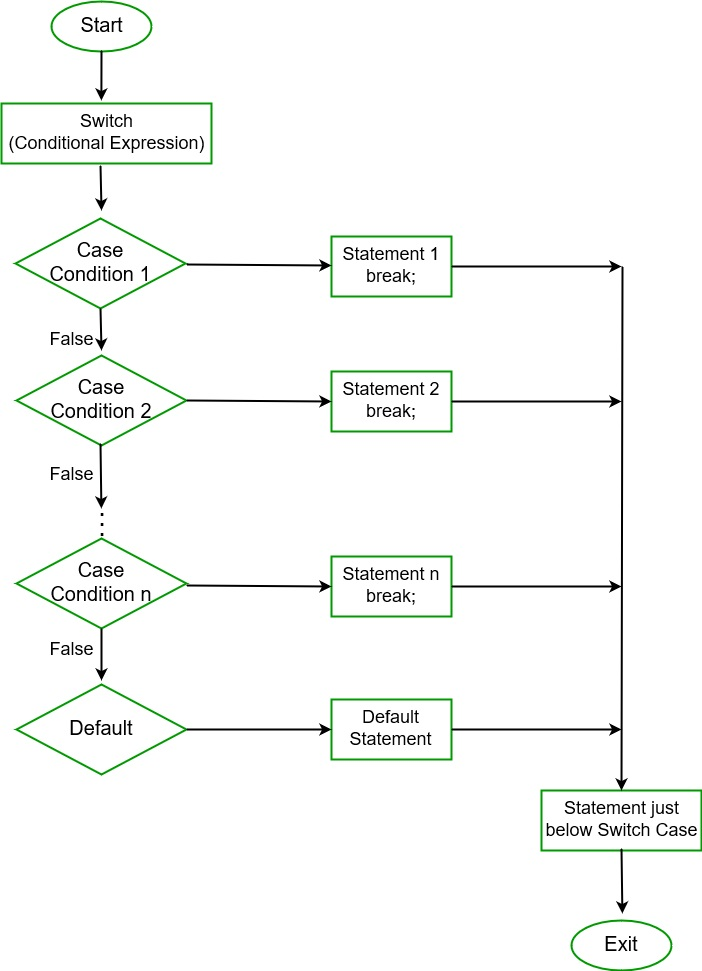Important points to remember:

• In C#, duplicate case values are not allowed.
• The data type of the variable in the switch and value of a case must be of the same type.
• The value of a case must be a constant or a literal. Variables are not allowed.
• The break in switch statement is used to terminate the current sequence.
• The default statement is optional and it can be used anywhere inside the switch statement.
• Multiple default statements are not allowed.

Example:

 `// C# program to illustrate``// switch case statement``using` `System;`` ` `public` `class` `GFG {`` ` `    ``// Main Method``    ``public` `static` `void` `Main(String[] args)``    ``{``        ``int` `nitem = 5;``        ``switch` `(nitem) {`` ` `        ``case` `1:``            ``Console.WriteLine(``"case 1"``);``            ``break``;`` ` `        ``case` `5:``            ``Console.WriteLine(``"case 5"``);``            ``break``;`` ` `        ``case` `9:``            ``Console.WriteLine(``"case 9"``);``            ``break``;`` ` `        ``default``:``            ``Console.WriteLine(``"No match found"``);``            ``break``;``        ``}``    ``}``}`
Output:
```case 5
```

To read more about this, you can refer to the article Switch Statement in C#.

### Loops

Looping in a programming language is a way to execute a statement or a set of statements multiple times depending on the result of the condition to be evaluated to execute statements. The result condition should be true to execute statements within loops. Loops are mainly divided into two categories:

• Entry Controlled Loops: The loops in which condition to be tested is present at the beginning of the loop body are known as Entry Controlled Loops. while loop and for loop are entry controlled loops.
• Exit Controlled Loops: The loops in which the testing condition is present at the end of the loop body are termed as Exit Controlled Loops. do-while is an exit controlled loop. In Exit Controlled Loops, the loop body will be evaluated for at least one time as the testing condition is present at the end of the loop body.

#### while Loop

he test condition is given in the beginning of the loop and all statements are executed till the given boolean condition satisfies when the condition becomes false, the control will be out from the while loop.

Syntax:

```while (boolean condition)
{
loop statements...
}```

Flowchart: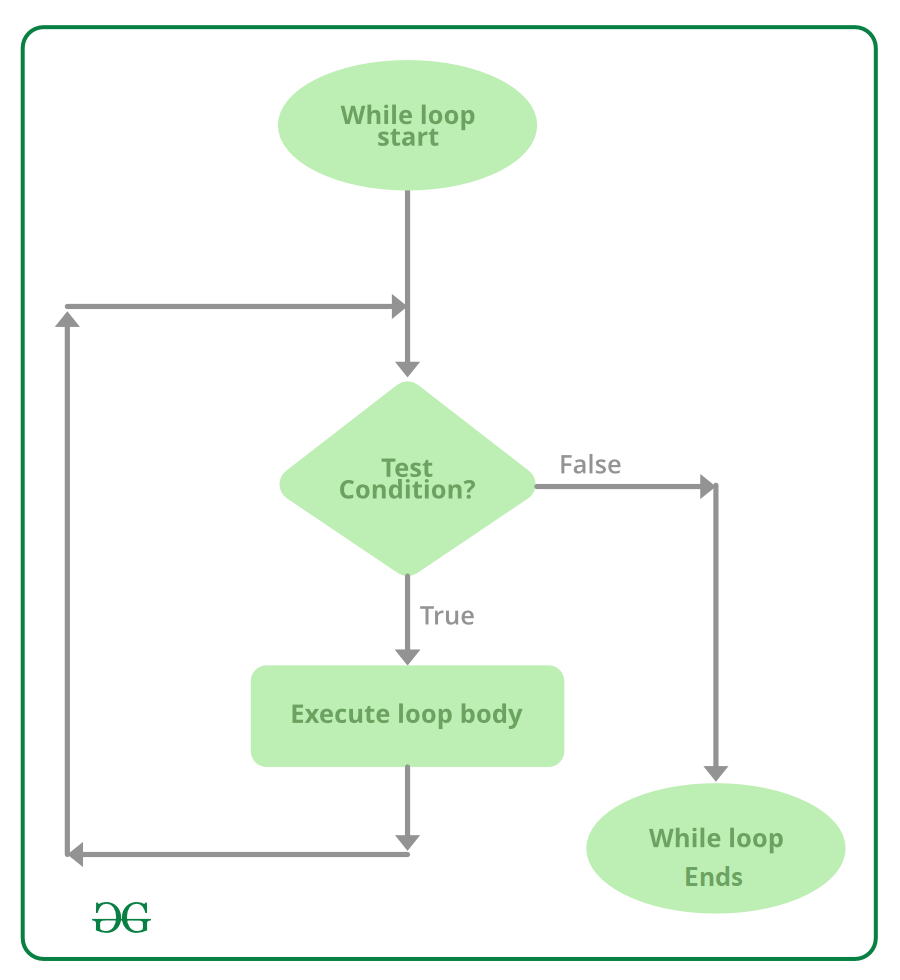Example:

 `// C# program to illustrate while loop``using` `System;`` ` `class` `whileLoopDemo {``    ``public` `static` `void` `Main()``    ``{``        ``int` `x = 1;`` ` `        ``// Exit when x becomes greater than 4``        ``while` `(x <= 4) {``            ``Console.WriteLine(``"GeeksforGeeks"``);`` ` `            ``// Increment the value of x for``            ``// next iteration``            ``x++;``        ``}``    ``}``}`

Output:

```GeeksforGeeks
GeeksforGeeks
GeeksforGeeks
GeeksforGeeks
```

#### do while Loop

do while loop is similar to while loop with the only difference that it checks the condition after executing the statements, i.e it will execute the loop body one time for sure because it checks the condition after executing the statements.

Syntax :

```do
{
statements..
}while (condition);
```

Flowchart: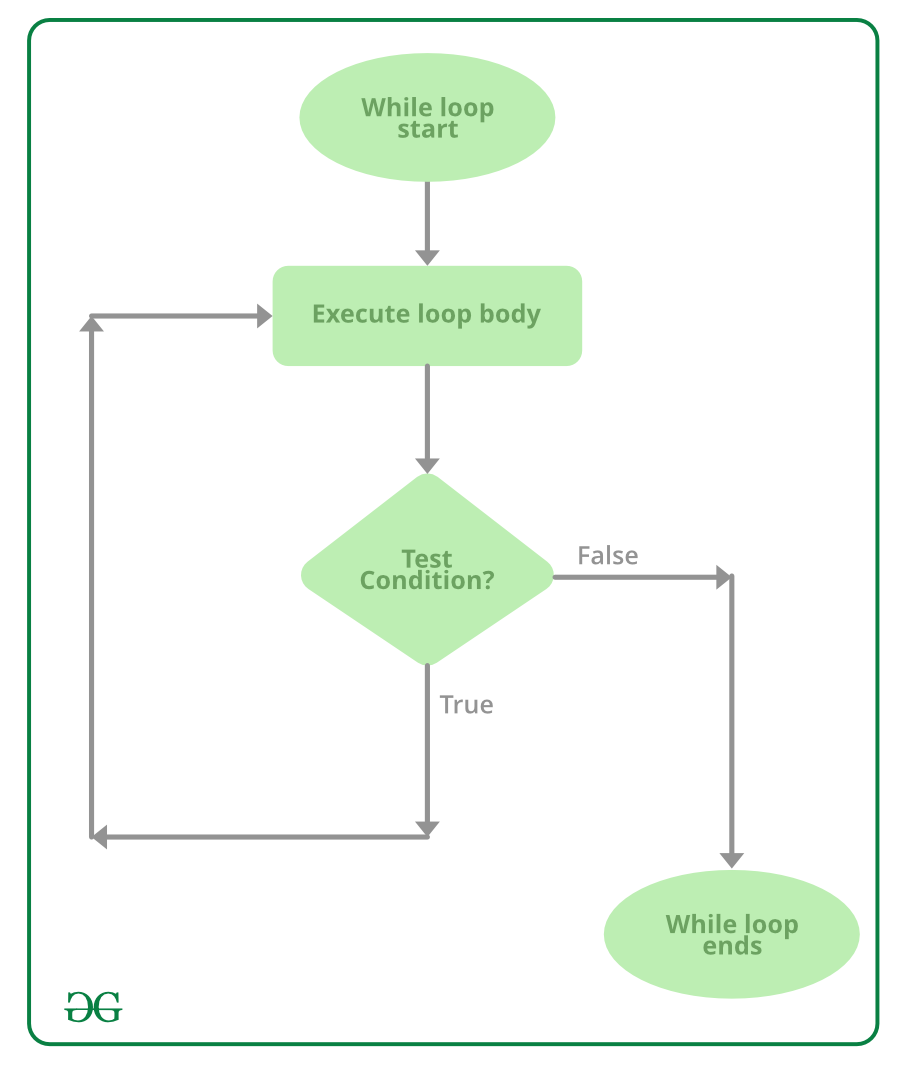Example:

 `// C# program to illustrate do-while loop``using` `System;`` ` `class` `dowhileloopDemo {``    ``public` `static` `void` `Main()``    ``{``        ``int` `x = 21;``        ``do` `{``            ``// The line will be printed even``            ``// if the condition is false``            ``Console.WriteLine(``"GeeksforGeeks"``);``            ``x++;``        ``} ``while` `(x < 20);``    ``}``}`

Output:

`GeeksforGeeks`

#### for Loop

for loop has similar functionality as while loop but with different syntax. for loops are preferred when the number of times loop statements are to be executed is known beforehand. The loop variable initialization, condition to be tested, and increment/decrement of the loop variable is done in one line in for loop thereby providing a shorter, easy to debug structure of looping.

```for (loop variable initialization ; testing condition;
increment / decrement)
{
// statements to be executed
}```

Flowchart: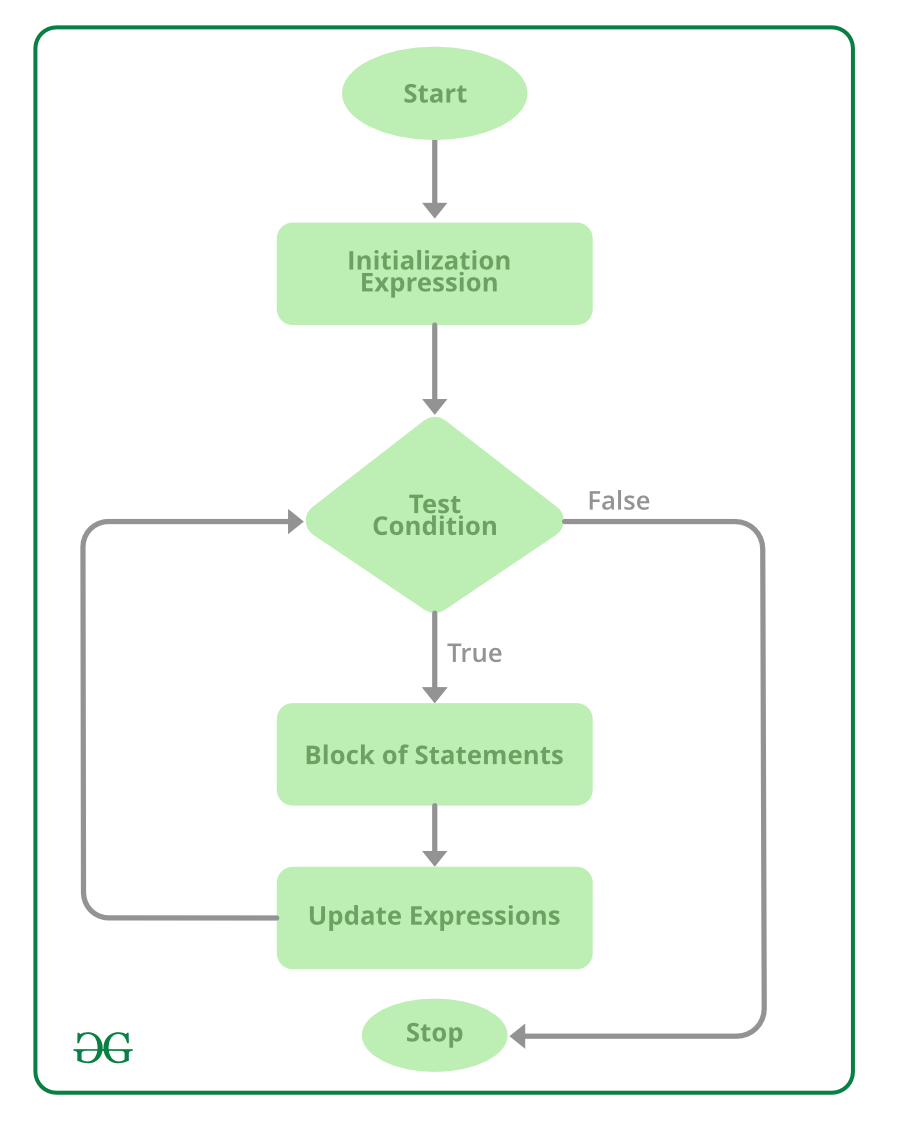1. Initialization of loop variable: Th expression / variable controlling the loop is initialized here. It is the starting point of for loop. An already declared variable can be used or a variable can be declared, local to loop only.

2. Testing Condition: The testing condition to execute statements of loop. It is used for testing the exit condition for a loop. It must return a boolean value true or false. When the condition became false the control will be out from the loop and for loop ends.

3. Increment / Decrement: The loop variable is incremented/decremented according to the requirement and the control then shifts to the testing condition again.

Note: Initialization part is evaluated only once when the for loop starts.

Example:

 `// C# program to illustrate for loop.``using` `System;`` ` `class` `forLoopDemo {``    ``public` `static` `void` `Main()``    ``{``        ``// for loop begins when x=1``        ``// and runs till x <=4``        ``for` `(``int` `x = 1; x <= 4; x++)``            ``Console.WriteLine(``"GeeksforGeeks"``);``    ``}``}`

Output:

```GeeksforGeeks
GeeksforGeeks
GeeksforGeeks
GeeksforGeeks
```

#### for each Loop

The foreach loop is used to iterate over the elements of the collection. The collection may be an array or a list. It executes for each element present in the array.

• It is necessary to enclose the statements of foreach loop in curly braces {}.
• Instead of declaring and initializing a loop counter variable, you declare a variable that is the same type as the base type of the array, followed by a colon, which is then followed by the array name.
• In the loop body, you can use the loop variable you created rather than using an indexed array element.

Syntax:

```foreach(data_type var_name in collection_variable)
{
// statements to be executed
}
```

Flowchart: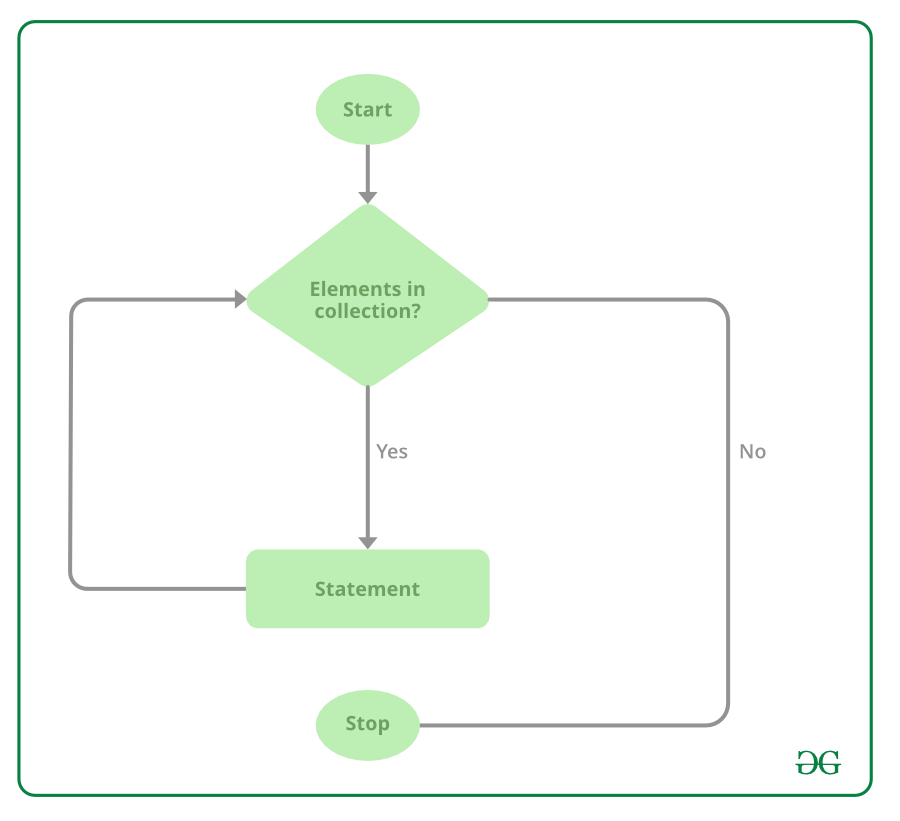Example:

 `// C# program to illustrate the``// use of foreach loop``using` `System;`` ` `class` `GFG {`` ` `    ``// Main Method``    ``static` `public` `void` `Main()``    ``{`` ` `        ``Console.WriteLine(``"Print array:"``);`` ` `        ``// creating an array``        ``int``[] a_array = ``new` `int``[] { 1, 2, 3, 4, 5, 6, 7 };`` ` `        ``// foreach loop begin``        ``// it will run till the``        ``// last element of the array``        ``foreach``(``int` `items ``in` `a_array)``        ``{``            ``Console.WriteLine(items);``        ``}``    ``}``}`
Output:
```Print array:
1
2
3
4
5
6
7
```

Explanation: foreach loop in above program is equivalent to:

```for(int items = 0; items < a_array.Length; items++)
{
Console.WriteLine(a_array[items]);
}
```

To know more about Loops you can refer to the articles

### Jump Statements

In C#, Jump statements are used to transfer control from one point to another point in the program due to some specified code while executing the program. There are mainly 4 keywords in the Jump Statements:

• break
• continue
• goto
• return

#### break statement

The break statement is used to terminate the loop or statement in which it present. After that, the control will pass to the statements that present after the break statement, if available. If the break statement present in the nested loop, then it terminates only those loops which contains break statement.

Flowchart: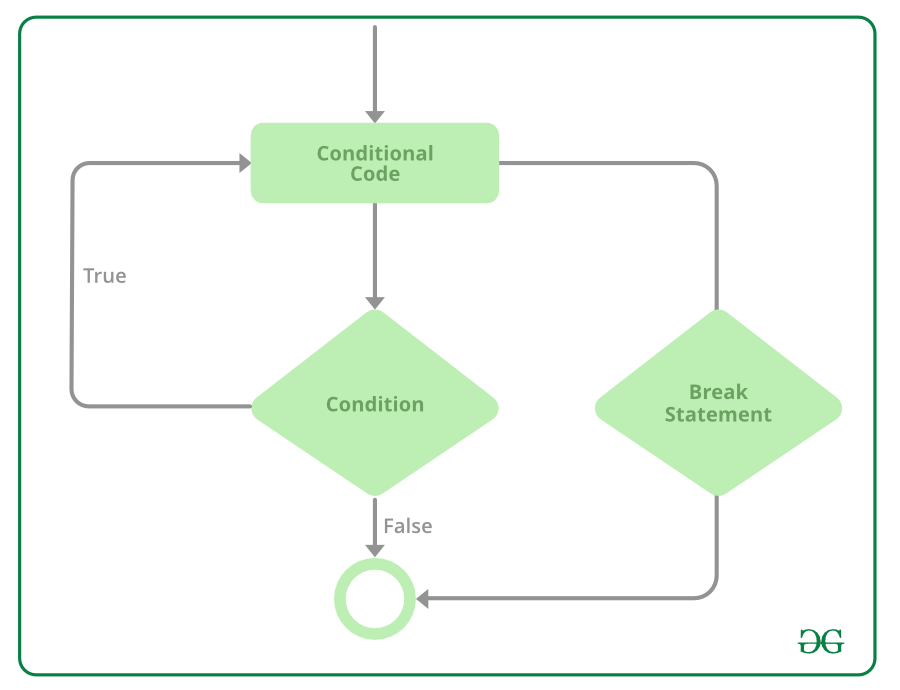Example:

 `// C# program to illustrate the``// use of break statement``using` `System;`` ` `class` `Geeks {`` ` `    ``// Main Method``    ``static` `public` `void` `Main()``    ``{`` ` `        ``// GeeksforGeeks is printed only 2 times``        ``// because of break statement``        ``for` `(``int` `i = 1; i < 4; i++) {``            ``if` `(i == 3)``                ``break``;`` ` `            ``Console.WriteLine(``"GeeksforGeeks"``);``        ``}``    ``}``}`
Output:
```GeeksforGeeks
GeeksforGeeks
```

#### continue statement

This statement is used to skip over the execution part of the loop on a certain condition. After that, it transfers the control to the beginning of the loop. Basically, it skips its following statements and continues with the next iteration of the loop.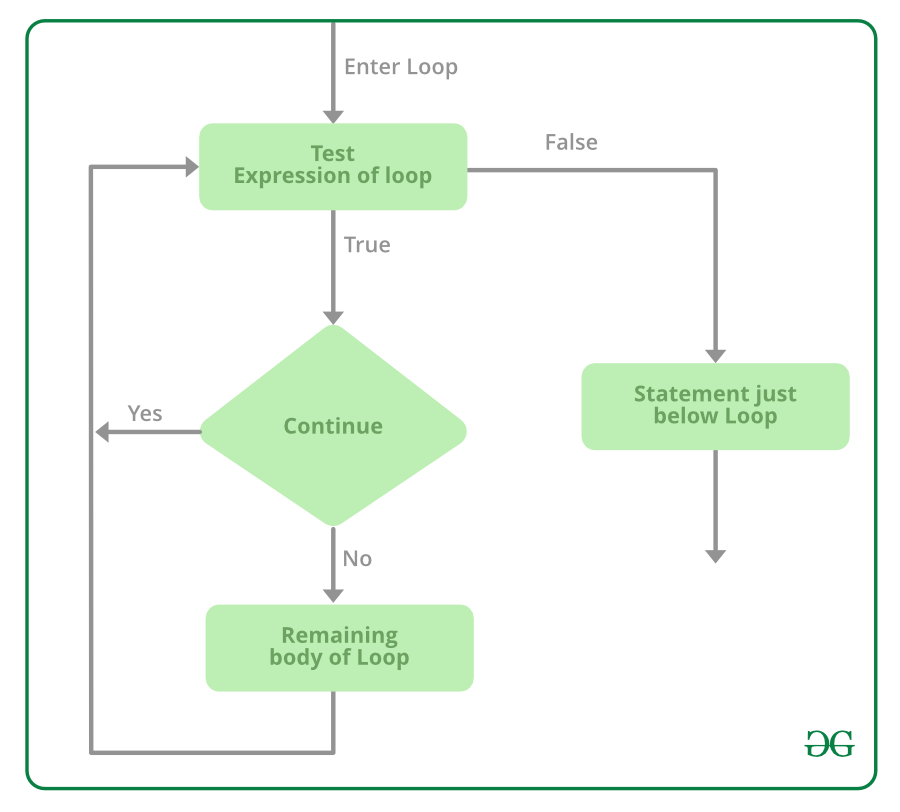Example:

 `// C# program to illustrate the``// use of continue statement``using` `System;`` ` `class` `Geeks {`` ` `    ``// Main Method``    ``public` `static` `void` `Main()``    ``{`` ` `        ``// This will skip 4 to print``        ``for` `(``int` `i = 1; i <= 10; i++) {`` ` `            ``// if the value of i becomes 4 then``            ``// it will skip 4 and send the``            ``// transfer to the for loop and``            ``// continue with 5``            ``if` `(i == 4)``                ``continue``;`` ` `            ``Console.WriteLine(i);``        ``}``    ``}``}`
Output:
```1
2
3
5
6
7
8
9
10
```

#### goto statement

This statement is used to transfer control to the labeled statement in the program. The label is the valid identifier and placed just before the statement from where the control is transferred.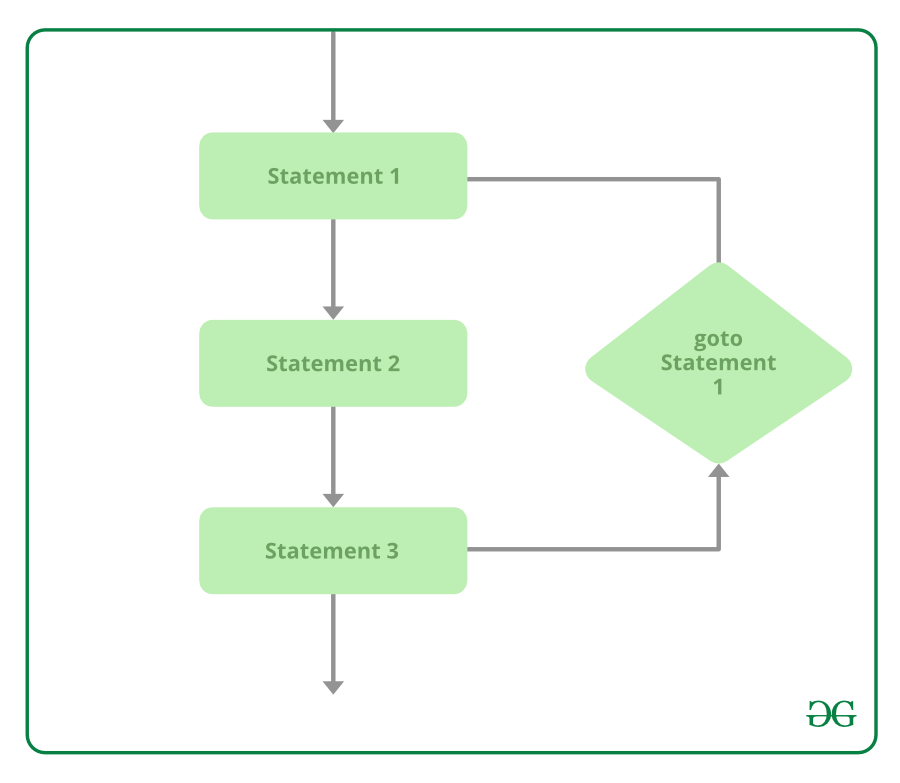Example:

 `// C# program to illustrate the``// use of goto statement``using` `System;`` ` `class` `Geeks {`` ` `    ``// Main Method``    ``static` `public` `void` `Main()``    ``{``        ``int` `number = 20;``        ``switch` `(number) {`` ` `        ``case` `5:``            ``Console.WriteLine(``"case 5"``);``            ``break``;``        ``case` `10:``            ``Console.WriteLine(``"case 10"``);``            ``break``;``        ``case` `20:``            ``Console.WriteLine(``"case 20"``);`` ` `            ``// goto statement transfer``            ``// the control to case 5``            ``goto` `case` `5;`` ` `        ``default``:``            ``Console.WriteLine(``"No match found"``);``            ``break``;``        ``}``    ``}``}`
Output:
```case 20
case 5
```

#### return statement

This statement terminates the execution of the method and returns the control to the calling method. It returns an optional value. If the type of method is void, then the return statement can be excluded.

Example:

 `// C# program to illustrate the``// use of return statement``using` `System;`` ` `class` `Geeks {`` ` `    ``// creating simple addition function``    ``static` `int` `Addition(``int` `a)``    ``{`` ` `        ``// add two value and``        ``// return the result of addition``        ``int` `add = a + a;`` ` `        ``// using return statement``        ``return` `add;``    ``}`` ` `    ``// Main Method``    ``static` `public` `void` `Main()``    ``{``        ``int` `number = 2;`` ` `        ``// calling addition function``        ``int` `result = Addition(number);``        ``Console.WriteLine(``"The addition is {0}"``, result);``    ``}``}`
Output:
```The addition is 4
```

To read more about this, you can refer to the article Jump Statements in C#

### Arrays

An array is a group of like-typed variables that are referred to by a common name. And each data item is called an element of the array. The data types of the elements may be any valid data type like char, int, float, etc. and the elements are stored in a contiguous location. Length of the array specifies the number of elements present in the array. In C# the allocation of memory for the arrays is done dynamically. And arrays are kind of objects, therefore it is easy to find their size using the predefined functions. The variables in the array are ordered and each has an index beginning from 0. Arrays in C# work differently than they do in C/C++. The following figure shows how array stores values sequentially :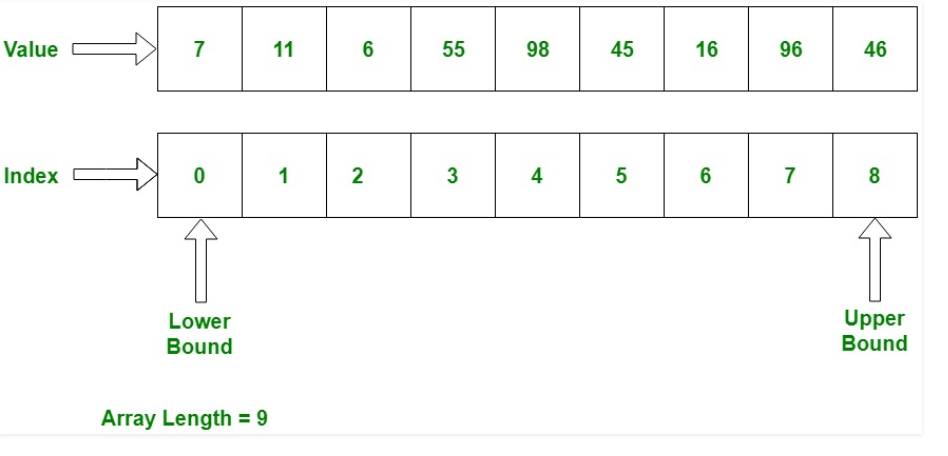Explanation: The index is starting from 0, which stores value. we can also store a fixed number of values in an array. Array index is to be increased by 1 in sequence whenever its not reach the array size.

Example:

 `// C# program to creating an array``// of the string as week days, store``// day values in the weekdays,``// and prints each value.``using` `System;``namespace` `geeksforgeeks {`` ` `class` `GFG {`` ` `    ``// Main Method``    ``public` `static` `void` `Main()``    ``{`` ` `        ``// declares a 1D Array of string.``        ``string``[] weekDays;`` ` `        ``// allocating memory for days.``        ``weekDays = ``new` `string``[] { ``"Sun"``, ``"Mon"``, ``"Tue"``, ``"Wed"``,``                                  ``"Thu"``, ``"Fri"``, ``"Sat"` `};`` ` `        ``// Displaying Elements of array``        ``foreach``(``string` `day ``in` `weekDays)``            ``Console.Write(day + ``" "``);``    ``}``}``}`

Output :

```Sun Mon Tue Wed Thu Fri Sat
```

To know more about this, you can refer to the article Arrays in C#

### String

In C#, string is a sequence of Unicode characters or an array of characters. The range of Unicode characters will be U+0000 to U+FFFF. The array of characters is also termed as the text. So the string is the representation of the text. A string is an important concept and people get confused about whether the string is a keyword or an object or a class. So let’s clear out this concept.

A string is represented by the class System.String. The “string” keyword is an alias for System.String class and instead of writing System.String one can use String which is a shorthand for System.String class. So we can say string and String both can be used as an alias of System.String class. So string is an object of System.String class.

Example:

string s1 = “GeeksforGeeks”; // creating the string using string keyword

String s2 = “GFG”; // creating the string using String class

System.String s3 = “Pro Geek”; // creating the string using String class

The String class is defined in the .NET base class library. In other words a String object is a sequential collection of System.Char objects which represents a string. The maximum size of String object in memory is 2GB or about 1 billion characters. System.String class is immutable, i.e once created its state cannot be altered.

Program: To illustrate how to declare the string and initialize the string. Also, below program show the declaration and initialization of a string in a single line.

 `// C# program to declare string using``// string, String and System.String``// and initialization of string``using` `System;``class` `Geeks {`` ` `    ``// Main Method``    ``static` `void` `Main(``string``[] args)``    ``{`` ` `        ``// declare a string Name using``        ``// "System.String" class``        ``System.String Name;`` ` `        ``// initialization of String``        ``Name = ``"Geek"``;`` ` `        ``// declare a string id using``        ``// using an alias(shorthand)``        ``// "String" of System.String``        ``// class``        ``String id;`` ` `        ``// initialization of String``        ``id = ``"33"``;`` ` `        ``// declare a string mrk using``        ``// string keyword``        ``string` `mrk;`` ` `        ``// initialization of String``        ``mrk = ``"97"``;`` ` `        ``// Declaration and initialization of``        ``// the string in a single line``        ``string` `rank = ``"1"``;`` ` `        ``// Displaying Result``        ``Console.WriteLine(``"Name: {0}"``, Name);``        ``Console.WriteLine(``"Id: {0}"``, id);``        ``Console.WriteLine(``"Marks: {0}"``, mrk);``        ``Console.WriteLine(``"Rank: {0}"``, rank);``    ``}``}`
Output:
```Name: Geek
Id: 33
Marks: 97
Rank: 1
```

To know more about this, you can refer to the article, String in C#

### Access Modifiers

Access Modifiers are keywords that define the accessibility of a member, class or datatype in a program. These are mainly used to restrict unwanted data manipulation by external programs or classes. There are 4 access modifiers (public, protected, internal, private) which defines the 6 accessibility levels as follows:

• public
• protected
• internal
• protected internal
• private
• private protected

The Accessibility table of these modifiers is given below:

publicprotectedinternalprotected internalprivateprivate protected
Entire programYesNoNoNoNoNo
Containing classYesYesYesYesYesYes
Current assemblyYesNoYesYesNoNo
Derived typesYesYesNoYesNoNo
Derived types within current assemblyYesYesNoYesNoYes

To know more about this, you can refer to the article Access Modifiers in C#

### Object-Oriented Programming

Object-oriented programming aims to implement real-world entities like inheritance, hiding, polymorphism, etc in programming. The main aim of OOP is to bind together the data and the functions that operate on them so that no other part of the code can access this data except that function.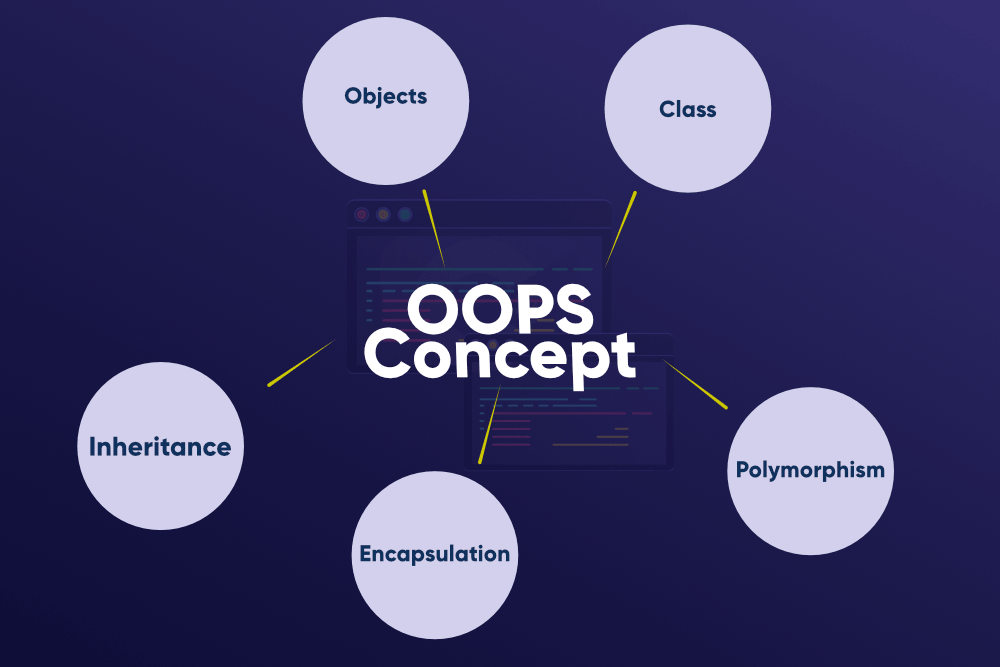#### Class and Object

Class and Object are the basic concepts of Object-Oriented Programming which revolve around the real-life entities. A class is a user-defined blueprint or prototype from which objects are created. Basically, a class combines the fields and methods(member function which defines actions) into a single unit. In C#, classes support polymorphism, inheritance and also provide the concept of derived classes and base classes.
Object is a basic unit of Object-Oriented Programming and represents the real-life entities. A typical C# program creates many objects, which as you know, interact by invoking methods.

Example:

 `// C# program to illustrate class and object concept``using` `System;`` ` `// Class Declaration``public` `class` `Dog {`` ` `    ``// Instance Variables``    ``String name;``    ``String breed;``    ``int` `age;``    ``String color;`` ` `    ``// Constructor Declaration of Class``    ``public` `Dog(String name, String breed,``               ``int` `age, String color)``    ``{``        ``this``.name = name;``        ``this``.breed = breed;``        ``this``.age = age;``        ``this``.color = color;``    ``}`` ` `    ``// Property 1``    ``public` `String getName()``    ``{``        ``return` `name;``    ``}`` ` `    ``// Property 2``    ``public` `String getBreed()``    ``{``        ``return` `breed;``    ``}`` ` `    ``// Property 3``    ``public` `int` `getAge()``    ``{``        ``return` `age;``    ``}`` ` `    ``// Property 4``    ``public` `String getColor()``    ``{``        ``return` `color;``    ``}`` ` `    ``// Method 1``    ``public` `String toString()``    ``{``        ``return` `(``"Hi my name is "` `+ ``this``.getName()``                ``+ ``".\nMy breed, age and color are "` `+ ``this``.getBreed()``                ``+ ``", "` `+ ``this``.getAge() + ``", "` `+ ``this``.getColor());``    ``}`` ` `    ``// Main Method``    ``public` `static` `void` `Main(String[] args)``    ``{`` ` `        ``// Creating object``        ``Dog tuffy = ``new` `Dog(``"tuffy"``, ``"papillon"``, 5, ``"white"``);``        ``Console.WriteLine(tuffy.toString());``    ``}``}`

Output:

```Hi my name is tuffy.
My breed, age, and color are papillon, 5, white
```

To know more about this, you can go through the article Class and Object in C#.

#### Constructors

A constructor is a special method of the class that gets automatically invoked whenever an instance of the class is created. Like methods, a constructor also contains the collection of instructions that are executed at the time of Object creation. It is used to assign initial values to the data members of the same class.

Example:

 `// C# Program to illustrate the constructors``using` `System;``namespace` `ParameterizedConstructorExample {`` ` `class` `Geek {`` ` `    ``// data members of the class.``    ``String name;``    ``int` `id;`` ` `    ``// parameterized constructor would``    ``// initialized data members with``    ``// the values of passed arguments``    ``// while object of that class created.``    ``Geek(String name, ``int` `id)``    ``{``        ``this``.name = name;``        ``this``.id = id;``    ``}`` ` `    ``// Main Method``    ``public` `static` `void` `Main()``    ``{`` ` `        ``// This will invoke parameterized``        ``// constructor.``        ``Geek geek1 = ``new` `Geek(``"GFG"``, 1);``        ``Console.WriteLine(``"GeekName = "` `+ geek1.name + ``" and GeekId = "` `+ geek1.id);``    ``}``}``}`

Output:

`GeekName = GFG and GeekId = 1`

To know more about constructors, you can refer to the article Constructors in C#.

#### Destructors

Destructors in C# are methods inside the class used to destroy instances of that class when they are no longer needed. The Destructor is called implicitly by the .NET Framework’s Garbage collector and therefore programmer has no control when to invoke the destructor. An instance variable or an object is eligible for destruction when it is no longer reachable.

Example:

 `// C# Program to illustrate how``// a destructor works``using` `System;`` ` `namespace` `GeeksforGeeks {`` ` `class` `Complex {`` ` `    ``// Class members, private``    ``// by default``    ``int` `real, img;`` ` `    ``// Defining the constructor``    ``public` `Complex()``    ``{``        ``real = 0;``        ``img = 0;``    ``}`` ` `    ``// SetValue method sets``    ``// value of real and img``    ``public` `void` `SetValue(``int` `r, ``int` `i)``    ``{``        ``real = r;``        ``img = i;``    ``}`` ` `    ``// DisplayValue displays``    ``// values of real and img``    ``public` `void` `DisplayValue()``    ``{``        ``Console.WriteLine(``"Real = "` `+ real);``        ``Console.WriteLine(``"Imaginary = "` `+ img);``    ``}`` ` `    ``// Defining the destructor``    ``// for class Complex``    ``~Complex()``    ``{``        ``Console.WriteLine(``"Destructor was called"``);``    ``}`` ` `} ``// End class Complex`` ` `// Driver Class``class` `Program {`` ` `    ``// Main Method``    ``static` `void` `Main(``string``[] args)``    ``{`` ` `        ``// Creating an instance of class``        ``// Complex C invokes constructor``        ``Complex C = ``new` `Complex();`` ` `        ``// Calling SetValue method using``        ``// instance C Setting values of``        ``// real to 2 and img to 3``        ``C.SetValue(2, 3);`` ` `        ``// Displaying values of real``        ``// and imaginary parts``        ``C.DisplayValue();`` ` `        ``// Instance is no longer needed``        ``// Destructor will be called`` ` `    ``} ``// End Main`` ` `} ``// End class Program``}`

Output:

```Real = 2
Imaginary = 3
Destructor was called
```

Explanation: In the above example, the class consists of a constructor Complex(), a SetValue method to set the value of the complex class’ instance, a DisplayValue method to display the value of the instance and a Destructor ~Complex() to destroy the object when the instance is no longer required, it prints the message “Destructor was called”, which depends on the programmer what message he/she wants to display or it can also be left blank.

To know more about destructors, you can refer to the article Destructors in C#.

#### Inheritance

Inheritance is an important pillar of OOP(Object Oriented Programming). It is the mechanism in C# by which one class is allowed to inherit the features(fields and methods) of another class.

Important terminology:

• Super Class: The class whose features are inherited is known as superclass(or a base class or a parent class).
• Sub Class: The class that inherits the other class is known as subclass(or a derived class, extended class, or child class). The subclass can add its own fields and methods in addition to the superclass fields and methods.
• Reusability: Inheritance supports the concept of “reusability”, i.e. when we want to create a new class and there is already a class that includes some of the code that we want, we can derive our new class from the existing class. By doing this, we are reusing the fields and methods of the existing class.

Example:

 `// C# program to illustrate the``// concept of inheritance``using` `System;``namespace` `ConsoleApplication1 {`` ` `// Base class``class` `GFG {`` ` `    ``// data members``    ``public` `string` `name;``    ``public` `string` `subject;`` ` `    ``// public method of base class``    ``public` `void` `readers(``string` `name, ``string` `subject)``    ``{``        ``this``.name = name;``        ``this``.subject = subject;``        ``Console.WriteLine(``"Myself: "` `+ name);``        ``Console.WriteLine(``"My Favorite Subject is: "` `+ subject);``    ``}``}`` ` `// inheriting the GFG class using :``class` `GeeksforGeeks : GFG {`` ` `    ``// constructor of derived class``    ``public` `GeeksforGeeks()``    ``{``        ``Console.WriteLine(``"GeeksforGeeks"``);``    ``}``}`` ` `// Driver class``class` `Sudo {`` ` `    ``// Main Method``    ``static` `void` `Main(``string``[] args)``    ``{`` ` `        ``// creating object of derived class``        ``GeeksforGeeks g = ``new` `GeeksforGeeks();`` ` `        ``// calling the method of base class``        ``// using the derived class object``        ``g.readers(``"Kirti"``, ``"C#"``);``    ``}``}``}`

Output:

```GeeksforGeeks
Myself: Kirti
My Favorite Subject is: C#
```

To know more about this, you can refer to the article Inheritance in C#.

#### Encapsulation

Encapsulation is defined as the wrapping up of data under a single unit. It is the mechanism that binds together code and the data it manipulates. In a different way, encapsulation is a protective shield that prevents the data from being accessed by the code outside this shield. Encapsulation can be achieved by declaring all the variables in the class as private and using C# Properties in the class to set and get the values of variables.

Example:

 `// C# program to illustrate encapsulation``using` `System;`` ` `public` `class` `DemoEncap {`` ` `    ``// private variables declared``    ``// these can only be accessed by``    ``// public methods of class``    ``private` `String studentName;``    ``private` `int` `studentAge;`` ` `    ``// using accessors to get and``    ``// set the value of studentName``    ``public` `String Name``    ``{`` ` `        ``get``        ``{``            ``return` `studentName;``        ``}`` ` `        ``set``        ``{``            ``studentName = value;``        ``}``    ``}`` ` `    ``// using accessors to get and``    ``// set the value of studentAge``    ``public` `int` `Age``    ``{`` ` `        ``get``        ``{``            ``return` `studentAge;``        ``}`` ` `        ``set``        ``{``            ``studentAge = value;``        ``}``    ``}``}`` ` `// Driver Class``class` `GFG {`` ` `    ``// Main Method``    ``static` `public` `void` `Main()``    ``{`` ` `        ``// creating object``        ``DemoEncap obj = ``new` `DemoEncap();`` ` `        ``// calls set accessor of the property Name,``        ``// and pass "Ankita" as value of the``        ``// standard field 'value'``        ``obj.Name = ``"Ankita"``;`` ` `        ``// calls set accessor of the property Age,``        ``// and pass "21" as value of the``        ``// standard field 'value'``        ``obj.Age = 21;`` ` `        ``// Displaying values of the variables``        ``Console.WriteLine(``"Name: "` `+ obj.Name);``        ``Console.WriteLine(``"Age: "` `+ obj.Age);``    ``}``}`

Output:

```Name: Ankita
Age: 21
```

Explanation: In the above program the class DemoEncap is encapsulated as the variables are declared as private. To access these private variables we are using the Name and Age accessors which contains the get and set method to retrieve and set the values of private fields. Accessors are defined as public so that they can access in other classes.
To know more about this, you can refer to the article Encapsulation in C#.

#### Polymorphism

Polymorphism refers to the ability of OOPs programming languages to differentiate between entities with the same name efficiently. This is done by Perl with the help of the signature and declaration of these entities.

Example:

```class base_class
{
public void gfg();
}

class derived_class : base_class
{
public void gfg();
}

class Main_Method
{
static void Main()
{
derived_class d = new derived_class();
d.gfg();
}
}
```

### Methods

Methods are generally the block of codes or statements in a program that gives the user the ability to reuse the same code which ultimately saves the excessive use of memory, acts as a time saver and more importantly, it provides a better readability of code. So basically, a method is a collection of statements that perform some specific task and return the result to the caller. A method can also perform some specific task without returning anything.

Example :

```// Method Name --> GetCircleArea()
// Return Type ---> double
static double GetCircleArea(double radius)
{
const float pi = 3.14F;
double area = pi * radius * radius;
return area;
}
```

#### Method Declaration

Method declaration means the way to construct method including its naming.

Syntax :

```<Access_Modifier> <return_type> <method_name>([<param_list>])
```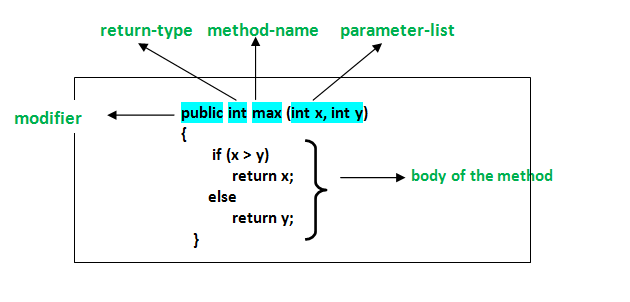In C# a method declaration consists of the following components as follows :

• Modifier : It defines access type of the method i.e. from where it can be accessed in your application. In C# there are Public, Protected, Private access modifiers.
• Name of the Method : It describes the name of the user defined method by which the user calls it or refer it. Eg. GetName()
• Return type: It defines the data type returned by the method. It depends upon user as it may also return void value i.e return nothing
• Body of the Method : It refers to the line of code of tasks to be performed by the method during its execution. It is enclosed between braces.
• Parameter list : Comma separated list of the input parameters are defined, preceded with their data type, within the enclosed parenthesis. If there are no parameters, then empty parentheses () have to use out.

Method Signature : Method Signature is defined by mainly two parameters(number of parameters, type of the parameters and order of the parameters), One of them is Method Name and second one is its Parameter list.

Method Naming : Name of a method or a function in any programming language whether in C++ or Java or C# holds great importance and is mainly used in order to call that method for its execution. For example, findSum, computeMax, setX and getX etc. There are certain pre-defined rules for naming methods which a user should follow :

• The method name must be some kind of Noun or a verb.
• It’s naming should be done in such a way that it must describe the purpose of that method.
• The first letter of the method name can be either a small letter or a Capital letter, however, it is recommended to use the capital one.

These rules are not mandatory, but recommendable. Generally, a method has a unique name within the class in which it is defined but sometime a method might have the same name as other method names within the same class as method overloading is allowed in C#.

The Method Body : As discussed above the body of the method consists of statements of code which a user wants to perform. After the method has been declared, it is dependent on the user whether to define its implementation or not. Not writing any implementation, makes the method not to perform any task. However, when the user wants to perform certain tasks using method then it must write the statements for execution in the body of the method. The below syntax describes the basic structure of the method body :

Syntax :

```<return_type> <method_name>(<parameter_list>)
{

// Implementation of the method code goes here.....

}

```

#### Method Calling

Method Invocation or Method Calling is done when the user wants to execute the method. The method needs to be called for using its functionality. A method returns to the code that invoked it when:

• It completes all the statements in the method
• It reaches a return statement
• Throws an exception

Example : In the code below, a method named Sum() is called.

 `// C# program to illustrate``// method calling``using` `System;``namespace` `ConsoleApplication1 {`` ` `class` `Geeks {`` ` `    ``// Here Sum() method asks for two``    ``// parameters from the user and``    ``// calculates the sum of these``    ``// and finally returns the result.``    ``static` `int` `Sum(``int` `x, ``int` `y)``    ``{`` ` `        ``// there are two local variables``        ``// 'a' and 'b' where 'a' is assigned``        ``// the value of parameter 'x' and``        ``// 'b' is assigned the value of``        ``// parameter 'y'``        ``int` `a = x;``        ``int` `b = y;`` ` `        ``// The local variable calculates``        ``// the sum of 'a' and 'b'``        ``// and returns the result``        ``// which is of 'int' type.``        ``int` `result = a + b;`` ` `        ``return` `result;``    ``}`` ` `    ``// Main Method``    ``static` `void` `Main(``string``[] args)``    ``{``        ``int` `a = 12;``        ``int` `b = 23;`` ` `        ``// Method Sum() is invoked and``        ``// the returned value is stored``        ``// in the local variable say 'c'``        ``int` `c = Sum(a, b);`` ` `        ``// Display Result``        ``Console.WriteLine(``"The Value of the sum is "` `+ c);``    ``}``}``}`

Output :

```The Value of the sum is 35
```

Refer to the below articles to know more about methods

#### Method Overloading

Method Overloading is a common way of implementing polymorphism. It is the ability to redefine a function in more than one form. A user can implement function overloading by defining two or more functions in a class sharing the same name. C# can distinguish the methods with different method signatures. i.e. the methods can have the same name but with different parameters list (i.e. the number of the parameters, order of the parameters, and data types of the parameters) within the same class.

Different ways of doing overloading methods- Method overloading can be done by changing:

1. The number of parameters in two methods.
2. The data types of the parameters of methods.
3. The Order of the parameters of methods.

Example:

 `// C# program to demonstrate the function``// overloading by changing the Number``// of parameters``using` `System;``class` `GFG {`` ` `    ``// adding two integer values.``    ``public` `int` `Add(``int` `a, ``int` `b)``    ``{``        ``int` `sum = a + b;``        ``return` `sum;``    ``}`` ` `    ``// adding three integer values.``    ``public` `int` `Add(``int` `a, ``int` `b, ``int` `c)``    ``{``        ``int` `sum = a + b + c;``        ``return` `sum;``    ``}`` ` `    ``// Main Method``    ``public` `static` `void` `Main(String[] args)``    ``{`` ` `        ``// Creating Object``        ``GFG ob = ``new` `GFG();`` ` `        ``int` `sum1 = ob.Add(1, 2);``        ``Console.WriteLine(``"sum of the two "``                          ``+ ``"integer value : "` `+ sum1);`` ` `        ``int` `sum2 = ob.Add(1, 2, 3);``        ``Console.WriteLine(``"sum of the three "``                          ``+ ``"integer value : "` `+ sum2);``    ``}``}`
Output:
```sum of the two integer value : 3
sum of the three integer value : 6
```

To know more about this, you can refer to the article C# Method Overloading.

#### Method Overriding

Method Overriding in C# is similar to the virtual function in C++. Method Overriding is a technique that allows the invoking of functions from another class (base class) in the derived class. Creating a method in the derived class with the same signature as a method in the base class is called method overriding.
In simple words, Overriding is a feature that allows a subclass or child class to provide a specific implementation of a method that is already provided by one of its super-classes or parent classes. When a method in a subclass has the same name, same parameters or signature and same return type(or sub-type) as a method in its super-class, then the method in the subclass is said to override the method in the super-class. Method overriding is one of the ways by which C# achieves Run Time Polymorphism(Dynamic Polymorphism).

The method that is overridden by an override declaration is called the overridden base method. An override method is a new implementation of a member that is inherited from a base class. The overridden base method must be virtual, abstract, or override.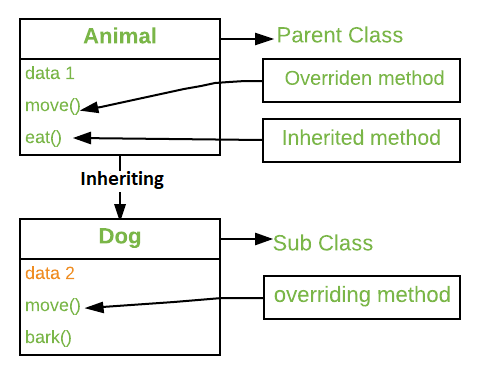Example:

 `// C# program to demonstrate the method overriding``// without using 'virtual' and 'override' modifiers``using` `System;`` ` `// base class name 'baseClass'``class` `baseClass`` ` `{``    ``public` `void` `show()``    ``{``        ``Console.WriteLine(``"Base class"``);``    ``}``}`` ` `// derived class name 'derived'``// 'baseClass' inherit here``class` `derived : baseClass {`` ` `    ``// overriding``    ``new` `public` `void` `show() {``        ``Console.WriteLine(``"Derived class"``);``    ``}``}`` ` `class` `GFG {`` ` `    ``// Main Method``    ``public` `static` `void` `Main()``    ``{`` ` `        ``// 'obj' is the object of``        ``// class 'baseClass'``        ``baseClass obj = ``new` `baseClass();`` ` `        ``// invokes the method 'show()'``        ``// of class 'baseClass'``        ``obj.show();`` ` `        ``obj = ``new` `derived();`` ` `        ``// it also invokes the method``        ``// 'show()' of class 'baseClass'``        ``obj.show();``    ``}``}`
Output:
```Base class
Base class
```

Explanation: In this program the object obj invokes class baseClass two times and call the method show() of class baseClass. To avoid this problem we use virtual and override keyword.
To know more about this, you can refer to the article C# Method Overriding.

#### Method Hiding

As we already know about polymorphism and method overriding in C#. C# also provides a concept to hide the methods of the base class from derived class, this concept is known as Method Hiding. It is also known as Method Shadowing. In method hiding, you can hide the implementation of the methods of a base class from the derived class using the new keyword. Or in other words, in method hiding, you can redefine the method of the base class in the derived class by using the new keyword.

Example:

 `// C# program to illustrate the``// concept of method hiding``using` `System;`` ` `// Base Class``public` `class` `My_Family {`` ` `    ``public` `void` `member()``    ``{``        ``Console.WriteLine(``"Total number of family members: 3"``);``    ``}``}`` ` `// Derived Class``public` `class` `My_Member : My_Family {`` ` `    ``// Reimplement the method of the base class``    ``// Using new keyword``    ``// It hides the method of the base class``    ``public` `new` `void` `member() {``        ``Console.WriteLine(``"Name: Rakesh, Age: 40 \nName: Somya, "``                          ``+ ``"Age: 39 \nName: Rohan, Age: 20 "``);``    ``}``}`` ` `// Driver Class``class` `GFG {`` ` `    ``// Main method``    ``static` `public` `void` `Main()``    ``{`` ` `        ``// Creating the object of the derived class``        ``My_Member obj = ``new` `My_Member();`` ` `        ``// Access the method of derived class``        ``obj.member();``    ``}``}`

Output:

```Name: Rakesh, Age: 40
Name: Somya, Age: 39
Name: Rohan, Age: 20
```

Explanation: In the above example, My_Family is the base class and My_Member is a derived class. In both the classes we have the same name method, i.e. member() method. But in the derived class, the member() method is declared with the new keyword. When this method is called, it prints the name and the age of the family members not the total number of family members. This means when we call the member() method with the help of the derived class object, it hides the same name method present in the base class due to the presence of the new keyword.

To know more about this, you can refer to the article C# Method Hiding.

### Collections

Collections standardize the way in which the objects are handled by your program. In other words, it contains a set of classes to contain elements in a generalized manner. With the help of collections, the user can perform several operations on objects like the store, update, delete, retrieve, search, sort, etc.

C# divide collection in several classes, some of the common classes are shown below: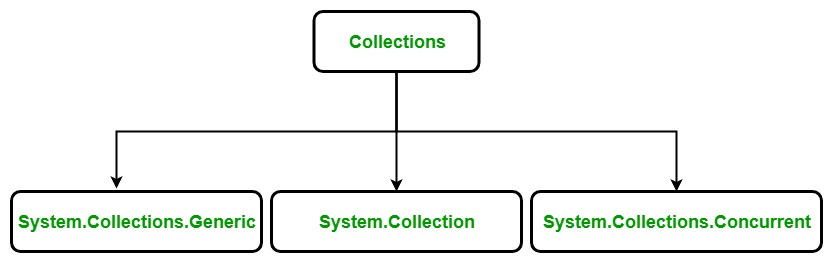Non-Generic collection in C# is defined in `System.Collections` namespace. It is a general-purpose data structure that works on object references, so it can handle any type of object, but not in a safe-type manner. Non-generic collections are defined by the set of interfaces and classes. To know more about this, you can refer to the article Collections in C#

The below table contains the frequently used classes of the `System.Collections` namespace:

Class nameDescription
ArrayListIt is a dynamic array means the size of the array is not fixed, it can increase and decrease at runtime.
HashtableIt represents a collection of key-and-value pairs that are organized based on the hash code of the key.
QueueIt represents a first-in, first out collection of objects. It is used when you need a first-in, first-out access of items.
StackIt is a linear data structure. It follows LIFO(Last In, First Out) pattern for Input/output.

#### ArrayList

ArrayList represents an ordered collection of an object that can be indexed individually. It is basically an alternative to an array. It also allows dynamic memory allocation, adding, searching and sorting items in the list.

Properties of ArrayList Class:

• Elements can be added or removed from the Array List collection at any point in time.
• The ArrayList is not guaranteed to be sorted.
• The capacity of an ArrayList is the number of elements the ArrayList can hold.
• Elements in this collection can be accessed using an integer index. Indexes in this collection are zero-based.
• It also allows duplicate elements.
• Using multidimensional arrays as elements in an ArrayList collection is not supported.

Example:

 `// C# code to create an ArrayList``using` `System;``using` `System.Collections;``using` `System.Collections.Generic;`` ` `class` `GFG {`` ` `    ``// Driver code``    ``public` `static` `void` `Main()``    ``{`` ` `        ``// Creating an ArrayList``        ``ArrayList myList = ``new` `ArrayList();`` ` `        ``// Adding elements to ArrayList``        ``myList.Add(``"Hello"``);``        ``myList.Add(``"World"``);`` ` `        ``Console.WriteLine(``"Count : "` `+ myList.Count);``        ``Console.WriteLine(``"Capacity : "` `+ myList.Capacity);``    ``}``}`

Output:

```Count : 2
Capacity : 4
```

#### Hashtable

The Hashtable class represents a collection of key/value pairs that are organized based on the hash code of the key. This class comes under the System.Collections namespace. The Hashtable class provides various types of methods that are used to perform different types of operation on the hashtables. In Hashtable, keys are used to access the elements present in the collection. For very large Hashtable objects, you can increase the maximum capacity to 2 billion elements on a 64-bit system.

Characteristics of Hashtable Class:

• In Hashtable, key cannot be null but value can be.
• In Hashtable, key objects must be immutable as long as they are used as keys in the Hashtable.
• The capacity of a Hashtable is the number of elements that Hashtable can hold.
• A hash function is provided by each key object in the Hashtable.

Example:

 `// C# program to create Hashtable``using` `System;``using` `System.Collections;`` ` `class` `GFG {`` ` `    ``// Main method``    ``static` `void` `Main(``string``[] args)``    ``{`` ` `        ``// create and initalize Hash table``        ``// using Add() method``        ``Hashtable g = ``new` `Hashtable();``        ``g.Add(1, ``"welcome"``);``        ``g.Add(2, ``"to"``);``        ``g.Add(3, ``"tutorials"``);``        ``g.Add(4, ``"of"``);``        ``g.Add(5, ``"C#"``);`` ` `        ``ICollection key = g.Keys;`` ` `        ``// Print Hash table``        ``Console.WriteLine(``"Hashtable:"``);``        ``Console.WriteLine();``        ``foreach``(``var` `val ``in` `key)``        ``{``            ``Console.WriteLine(val + ``"-"` `+ g[val]);``        ``}``    ``}``}`

Output:

```Hashtable:

5-C#
4-of
3-tutorials
2-to
1-welcome
```

#### Stack

Stack represents a last-in, first out collection of object. It is used when you need a last-in, first-out access to items. When you add an item in the list, it is called pushing the item and when you remove it, it is called popping the item. This class comes under System.Collections namespace.

Characteristics of Stack Class:

• The capacity of a Stack is the number of elements the Stack can hold. As elements are added to a Stack, the capacity is automatically increased as required through reallocation.
• If Count is less than the capacity of the stack, Push is an O(1) operation. If the capacity needs to be increased to accommodate the new element, Push becomes an O(n) operation, where n is Count. Pop is an O(1) operation.
• Stack accepts null as a valid value and allows duplicate elements.

Example:

 `// C# code to create a Stack``using` `System;``using` `System.Collections;``class` `GFG {`` ` `    ``// Driver code``    ``public` `static` `void` `Main()``    ``{`` ` `        ``// Creating a Stack``        ``Stack myStack = ``new` `Stack();`` ` `        ``// Inserting the elements into the Stack``        ``myStack.Push(``"1st Element"``);``        ``myStack.Push(``"2nd Element"``);``        ``myStack.Push(``"3rd Element"``);``        ``myStack.Push(``"4th Element"``);``        ``myStack.Push(``"5th Element"``);``        ``myStack.Push(``"6th Element"``);`` ` `        ``// Displaying the count of elements``        ``// contained in the Stack``        ``Console.Write(``"Total number of elements in the Stack are : "``);`` ` `        ``Console.WriteLine(myStack.Count);`` ` `        ``// Displaying the top element of Stack``        ``// without removing it from the Stack``        ``Console.WriteLine(``"Element at the top is : "` `+ myStack.Peek());`` ` `        ``// Displaying the top element of Stack``        ``// without removing it from the Stack``        ``Console.WriteLine(``"Element at the top is : "` `+ myStack.Peek());`` ` `        ``// Displaying the count of elements``        ``// contained in the Stack``        ``Console.Write(``"Total number of elements in the Stack are : "``);`` ` `        ``Console.WriteLine(myStack.Count);``    ``}``}`

Output:

```Total number of elements in the Stack are : 6
Element at the top is : 6th Element
Element at the top is : 6th Element
Total number of elements in the Stack are : 6
```

#### Queue

Queue represents a first-in, first out collection of object. It is used when you need a first-in, first-out access of items. When you add an item in the list, it is called enqueue, and when you remove an item, it is called dequeue . This class comes under System.Collections namespace and implements ICollection, IEnumerable, and ICloneable interfaces.

Characteristics of Queue Class:

• Enqueue adds an element to the end of the Queue.
• Dequeue removes the oldest element from the start of the Queue.
• Peek returns the oldest element that is at the start of the Queue but does not remove it from the Queue.
• The capacity of a Queue is the number of elements the Queue can hold.
• As elements are added to a Queue, the capacity is automatically increased as required by reallocating the internal array.
• Queue accepts null as a valid value for reference types and allows duplicate elements.

Example:

 `// C# code to create a Queue``using` `System;``using` `System.Collections;`` ` `class` `GFG {`` ` `    ``// Driver code``    ``public` `static` `void` `Main()``    ``{`` ` `        ``// Creating a Queue``        ``Queue myQueue = ``new` `Queue();`` ` `        ``// Inserting the elements into the Queue``        ``myQueue.Enqueue(``"one"``);`` ` `        ``// Displaying the count of elements``        ``// contained in the Queue``        ``Console.Write(``"Total number of elements in the Queue are : "``);`` ` `        ``Console.WriteLine(myQueue.Count);`` ` `        ``myQueue.Enqueue(``"two"``);`` ` `        ``// Displaying the count of elements``        ``// contained in the Queue``        ``Console.Write(``"Total number of elements in the Queue are : "``);`` ` `        ``Console.WriteLine(myQueue.Count);`` ` `        ``myQueue.Enqueue(``"three"``);`` ` `        ``// Displaying the count of elements``        ``// contained in the Queue``        ``Console.Write(``"Total number of elements in the Queue are : "``);`` ` `        ``Console.WriteLine(myQueue.Count);`` ` `        ``myQueue.Enqueue(``"four"``);`` ` `        ``// Displaying the count of elements``        ``// contained in the Queue``        ``Console.Write(``"Total number of elements in the Queue are : "``);`` ` `        ``Console.WriteLine(myQueue.Count);`` ` `        ``myQueue.Enqueue(``"five"``);`` ` `        ``// Displaying the count of elements``        ``// contained in the Queue``        ``Console.Write(``"Total number of elements in the Queue are : "``);`` ` `        ``Console.WriteLine(myQueue.Count);`` ` `        ``myQueue.Enqueue(``"six"``);`` ` `        ``// Displaying the count of elements``        ``// contained in the Queue``        ``Console.Write(``"Total number of elements in the Queue are : "``);`` ` `        ``Console.WriteLine(myQueue.Count);``    ``}``}`
Output:
```Total number of elements in the Queue are : 1
Total number of elements in the Queue are : 2
Total number of elements in the Queue are : 3
Total number of elements in the Queue are : 4
Total number of elements in the Queue are : 5
Total number of elements in the Queue are : 6
```

### Properties

Before going on properties lets have a look at why the concept of properties came into C#? The is because of two reasons:

• If the members of a class are private then how another class in C# will be able to read, write, or compute the value that field.
• If the members of the class are public then another class may misuse that member.

Properties are the special type of class members that provides a flexible mechanism to read, write, or compute the value of a private field. Properties can be used as if they are public data members, but they are actually special methods called accessors. This enables data to be accessed easily and helps to promote the flexibility and safety of methods. Encapsulation and hiding of information can also be achieved using properties. It uses pre-defined methods which are “get” and “set” methods which help to access and modify the properties.

Accessors: The block of “set” and “get” is known as “Accessors”. It is very essential to restrict the accessibility of property. There are two type of accessors i.e. get accessors and set accessors. There are different types of properties based on the “get” and set accessors:

• Read and Write Properties: When property contains both get and set methods.
• Read-Only Properties: When property contains only get method.
• Write Only Properties: When property contains only set method.
• Auto Implemented Properties: When there is no additional logic in the property accessors and it introduce in C# 3.0.

The syntax for Defining Properties:

```<access_modifier> <return_type> <property_name>
{
get { // body }
set { // body }
}
```

Where, <access_modifier> can be public, private, protected or internal. <return_type> can be any valid C# type. <property_name> can be user-defined.Properties can be different access modifiers like public, private, protected, internal. Access modifiers define how users of the class can access the property. The get and set accessors for the same property may have different access modifiers. A property may be declared as a static property by using the static keyword or may be marked as a virtual property by using the virtual keyword.

• Get Accessor: It specifies that the value of a field can access publicly. It returns a single value and it specifies the read-only property.
• Set Accessor: It will specify the assignment of a value to a private field in a property. It returns a single value and it specifies the write-only property.

Example:

 `// C# program to illustrate the``// read and wirte property``using` `System;`` ` `public` `class` `Student {`` ` `    ``// Declare name field``    ``private` `string` `name = ``"GeeksforGeeks"``;`` ` `    ``// Declare name property``    ``public` `string` `Name``    ``{`` ` `        ``get``        ``{``            ``return` `name;``        ``}`` ` `        ``set``        ``{``            ``name = value;``        ``}``    ``}``}`` ` `class` `TestStudent {`` ` `    ``// Main Method``    ``public` `static` `void` `Main(``string``[] args)``    ``{``        ``Student s = ``new` `Student();`` ` `        ``// calls set accessor of the property Name,``        ``// and pass "GFG" as value of the``        ``// standard field 'value'.``        ``s.Name = ``"GFG"``;`` ` `        ``// displays GFG, Calls the get accessor``        ``// of the property Name.``        ``Console.WriteLine(``"Name: "` `+ s.Name);``    ``}``}`

Output:

`Name: GFG`

To read more about this, you can refer to the article Properties in C#

### Indexers

An indexer allows an instance of a class or struct to be indexed as an array. If the user will define an indexer for a class, then the class will behave like a virtual array. Array access operator i.e ([ ]) is used to access the instance of the class which uses an indexer. A user can retrieve or set the indexed value without pointing an instance or a type member. Indexers are almost similar to the Properties. The main difference between Indexers and Properties is that the accessors of the Indexers will take parameters.

Syntax:

```[access_modifier] [return_type] this [argument_list]
{
get
{
// get block code
}
set
{
// set block code
}

}
```

In the above syntax:

• access_modifier: It can be public, private, protected or internal.
• return_type: It can be any valid C# type.
• this: It is the keyword which points to the object of the current class.
• argument_list: This specifies the parameter list of the indexer.
• get{ } and set { }: These are the accessors.

Example:

 `// C# program to illustrate the Indexer``using` `System;`` ` `// class declaration``class` `IndexerCreation {`` ` `    ``// class members``    ``private` `string``[] val = ``new` `string``;`` ` `    ``// Indexer declaration``    ``// Here pubic is the modifier``    ``// string is the return type of``    ``// Indexer and "this" is the keyword``    ``// having parameters list``    ``public` `string` `this``[``int` `index]``    ``{`` ` `        ``// get Accessor``        ``// retrieving the values``        ``// stored in val[] array``        ``// of strings``        ``get``        ``{`` ` `            ``return` `val[index];``        ``}`` ` `        ``// set Accessor``        ``// setting the value at``        ``// passed index of val``        ``set``        ``{`` ` `            ``// value keyword is used``            ``// to define the value``            ``// being assigned by the``            ``// set indexer.``            ``val[index] = value;``        ``}``    ``}``}`` ` `// Driver Class``class` `main {`` ` `    ``// Main Method``    ``public` `static` `void` `Main()``    ``{`` ` `        ``// creating an object of parent class which``        ``// acts as primary address for using Indexer``        ``IndexerCreation ic = ``new` `IndexerCreation();`` ` `        ``// Inserting values in ic[]``        ``// Here we are using the object``        ``// of class as an array``        ``ic = ``"C"``;``        ``ic = ``"CPP"``;``        ``ic = ``"CSHARP"``;`` ` `        ``Console.Write(``"Printing values stored in objects used as arrays\n"``);`` ` `        ``// printing values``        ``Console.WriteLine(``"First value = {0}"``, ic);``        ``Console.WriteLine(``"Second value = {0}"``, ic);``        ``Console.WriteLine(``"Third value = {0}"``, ic);``    ``}``}`

Output:

```Printing values stored in objects used as arrays
First value = C
Second value = CPP
Third value = CSHARP
```

To read more about this, you can refer to the article Indexers in C#

### Interface

To read more about this, you can refer to the article Interface in C#

Like a class, Interface can have methods, properties, events, and indexers as its members. But interfaces will contain only the declaration of the members. The implementation of the interface’s members will be given by class who implements the interface implicitly or explicitly.

• Interfaces specify what a class must do and not how.
• Interfaces can’t have private members.
• By default all the members of Interface are public and abstract.
• The interface will always defined with the help of keyword ‘interface‘.
• Interface cannot contain fields because they represent a particular implementation of data.
• Multiple inheritance is possible with the help of Interfaces but not with classes.

Syntax for Interface Declaration:

```interface  <interface_name >
{
// declare Events
// declare indexers
// declare methods
// declare properties
}
```

Syntax for Implementing Interface:

```class class_name : interface_name
```

To declare an interface, use interface keyword. It is used to provide total abstraction. That means all the members in the interface are declared with the empty body and are public and abstract by default. A class that implements an interface must implement all the methods declared in the interface.

Example:

 `// C# program to demonstrate working of``// interface``using` `System;`` ` `// A simple interface``interface` `inter1 {``    ``// method having only declaration``    ``// not definition``    ``void` `display();``}`` ` `// A class that implements interface.``class` `testClass : inter1 {`` ` `    ``// providing the body part of function``    ``public` `void` `display()``    ``{``        ``Console.WriteLine(``"Sudo Placement GeeksforGeeks"``);``    ``}`` ` `    ``// Main Method``    ``public` `static` `void` `Main(String[] args)``    ``{`` ` `        ``// Creating object``        ``testClass t = ``new` `testClass();`` ` `        ``// calling method``        ``t.display();``    ``}``}`
Output:
```Sudo Placement GeeksforGeeks
```

### Multithreading

Multi-threading is a process that contains multiple threads within a single process. Here each thread performs different activities. For example, we have a class and this call contains two different methods, now using multithreading each method is executed by a separate thread. So the major advantage of multithreading is it works simultaneously, which means multiple tasks execute at the same time. And also maximizing the utilization of the CPU because multithreading works on time-sharing concept mean each thread takes its own time for execution and does not affect the execution of another thread, this time interval is given by the operating system.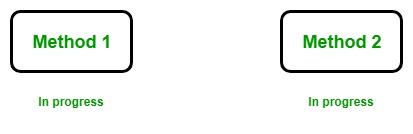Example:

 `// C# program to illustrate the``// concept of multithreading``using` `System;``using` `System.Threading;`` ` `public` `class` `GFG {`` ` `    ``// static method one``    ``public` `static` `void` `method1()``    ``{`` ` `        ``// It prints numbers from 0 to 10``        ``for` `(``int` `I = 0; I <= 10; I++) {``            ``Console.WriteLine(``"Method1 is : {0}"``, I);`` ` `            ``// When the value of I is equal to 5 then``            ``// this method sleeps for 6 seconds``            ``if` `(I == 5) {``                ``Thread.Sleep(6000);``            ``}``        ``}``    ``}`` ` `    ``// static method two``    ``public` `static` `void` `method2()``    ``{``        ``// It prints numbers from 0 to 10``        ``for` `(``int` `J = 0; J <= 10; J++) {``            ``Console.WriteLine(``"Method2 is : {0}"``, J);``        ``}``    ``}`` ` `    ``// Main Method``    ``static` `public` `void` `Main()``    ``{`` ` `        ``// Creating and initializing threads``        ``Thread thr1 = ``new` `Thread(method1);``        ``Thread thr2 = ``new` `Thread(method2);``        ``thr1.Start();``        ``thr2.Start();``    ``}``}`

Output :

```Method1 is : 0
Method1 is : 1
Method1 is : 2
Method1 is : 3
Method2 is : 0
Method2 is : 1
Method2 is : 2
Method2 is : 3
Method2 is : 4
Method2 is : 5
Method2 is : 6
Method2 is : 7
Method2 is : 8
Method2 is : 9
Method2 is : 10
Method1 is : 4
Method1 is : 5
Method1 is : 6
Method1 is : 7
Method1 is : 8
Method1 is : 9
Method1 is : 10
```

Explanation: Here, we create and initialize two threads, i.e thr1 and thr2 using Thread class. Now using `thr1.Start();` and `thr2.Start();` we start the execution of both the threads. Now both thread runs simultaneously and the processing of thr2 does not depend upon the processing of thr1 like in the single threaded model.

Note: Output may vary due to context switching.

To read more about this, you can refer to the article C# Multithreading.

### Regular Expression

In C#, Regular Expression is a pattern which is used to parse and check whether the given input text is matching with the given pattern or not. In C#, Regular Expressions are generally termed as C# Regex. The .Net Framework provides a regular expression engine that allows the pattern matching. Patterns may consist of any character literals, operators or constructors.
C# provides a class termed as Regex which can be found in System.Text.RegularExpression namespace. This class will perform two things:

• Parsing the inputing text for the regular expression pattern.
• Identify the regular expression pattern in the given text.

Example: Below example demonstrate the use of regex in Mobile Number Verification. Suppose you are making a form where you need to verify the user-entered mobile number then you can use regex.

 `// C# program to validate the Mobile``// Number using Regular Expressions``using` `System;``using` `System.Text.RegularExpressions;`` ` `class` `GFG {`` ` `    ``// Main Method``    ``static` `void` `Main(``string``[] args)``    ``{``        ``// Input strings to Match``        ``// valid mobile number``        ``string``[] str = { ``"9925612824"``,``                         ``"8238783138"``, ``"02812451830"` `};`` ` `        ``foreach``(``string` `s ``in` `str)``        ``{``            ``Console.WriteLine(``"{0} {1} a valid mobile number."``, s,``                              ``isValidMobileNumber(s) ? ``"is"` `: ``"is not"``);``        ``}`` ` `        ``Console.ReadKey();``    ``}`` ` `    ``// method containing the regex``    ``public` `static` `bool` `isValidMobileNumber(``string` `inputMobileNumber)``    ``{``        ``string` `strRegex = ``@"(^[0-9]{10}\$)|(^\+[0-9]{2}\s+[0-9]``        ``{ 2 }[0 - 9]``        ``{``            ``8``        ``}\$)|(^[0-9]{3}-[0-9]{4}-[0-9]{``            ``4}\$)"``;``         ` `        ``// Class Regex Repesents an``        ``// immutable regular expression.``        ``// Format                Pattern``        ``// xxxxxxxxxx           ^[0 - 9]{ 10}\$``        ``// +xx xx xxxxxxxx     ^\+[0 - 9]{ 2}\s +[0 - 9]{ 2}\s +[0 - 9]{ 8}\$``        ``// xxx - xxxx - xxxx   ^[0 - 9]{ 3} -[0 - 9]{ 4}-[0 - 9]{ 4}\$``        ``Regex re = ``new` `Regex(strRegex);`` ` `        ``// The IsMatch method is used to validate``        ``// a string or to ensure that a string``        ``// conforms to a particular pattern.``        ``if` `(re.IsMatch(inputMobileNumber))``            ``return` `(``true``);``        ``else``            ``return` `(``false``);``    ``}``}`

Output:

```9925612824 is a valid mobile number.
8238783138 is a valid mobile number.
02812451830 is not a valid mobile number.
```

To read more about this, you can refer to the article Regular Expressions.

### Exception Handling

An exception is defined as an event that occurs during the execution of a program that is unexpected by the program code. The actions to be performed in case of occurrence of an exception is not known to the program. In such a case, we create an exception object and call the exception handler code. The execution of an exception handler so that the program code does not crash is called exception handling. Exception handling is important because it gracefully handles an unwanted event, an exception so that the program code still makes sense to the user.

KeywordDefinition
tryUsed to define a try block. This block holds the code that may throw an exception.
catchUsed to define a catch block. This block catches the exception thrown by the try block.
finallyUsed to define the finally block. This block holds the default code.
throwUsed to throw an exception manually.

Let us take a simple example to understand what an exception is:

 `// C# program to show how``// Exceptions occur in a program``using` `System;`` ` `class` `GFG {`` ` `    ``static` `void` `Main(``string``[] args)``    ``{``        ``// Declare an array of max index 4``        ``int``[] arr = { 1, 2, 3, 4, 5 };`` ` `        ``// Display values of array elements``        ``for` `(``int` `i = 0; i < arr.Length; i++) {``            ``Console.WriteLine(arr[i]);``        ``}`` ` `        ``// Try to access invalid index of array``        ``Console.WriteLine(arr);``        ``// An execption is thrown upon executing``        ``// the above line``    ``}``}`

Runtime Error:

Unhandled Exception:
System.IndexOutOfRangeException: Index was outside the bounds of the array.
at GFG.Main (System.String[] args) [0x0002e] in <9fa39b3b4dec49eb8af89dc70d5a0618>:0
[ERROR] FATAL UNHANDLED EXCEPTION: System.IndexOutOfRangeException: Index was outside the bounds of the array.
at GFG.Main (System.String[] args) [0x0002e] in <9fa39b3b4dec49eb8af89dc70d5a0618>:0

Output:

```1
2
3
4
5
```

In the code given above, the array named ‘arr’ is defined for 5 elements, indices 0 to 4. When we try to access the 7th element of the array, that is non-existent, program code throws an exception and the above message is displayed. The exception can be handled using the System.Exception class of C#. This will be depicted in the code given below.

Exception Handling Using try-catch block

The code given below shows how we can handle exceptions using the try-catch block. The code that may generate an exception is placed inside the try block. In this case, the access to the 7th element is put inside the try block. When that statement is executed an exception is generated, which is caught by the catch block. The object of the type IndexOutOfRangeException is used to display a message to the user about the exception that has occurred.

Syntax:

```try
{
// statements that may cause an exception
}
catch( Exception obj)
{
// handler code
}```
 `// Exception handling of above code``// using try catch blocks`` ` `using` `System;`` ` `class` `Program : System.Exception {``    ``static` `void` `Main(``string``[] args)``    ``{``        ``// Declare an array of max index 4``        ``int``[] arr = { 1, 2, 3, 4, 5 };`` ` `        ``// Display values of array elements``        ``for` `(``int` `i = 0; i < arr.Length; i++) {``            ``Console.WriteLine(arr[i]);``        ``}`` ` `        ``try` `{`` ` `            ``// Try to access invalid index of array``            ``Console.WriteLine(arr);``            ``// An execption is thrown upon executing``            ``// the above line``        ``}``        ``catch` `(IndexOutOfRangeException e) {`` ` `            ``// The Message property of the object``            ``// of type IndexOutOfRangeException``            ``// is used to display the type of exception``            ``// that has occurred to the user.``            ``Console.WriteLine(``"An Exception has occurred : {0}"``, e.Message);``        ``}``    ``}``}`

Output:

```1
2
3
4
5
An Exception has occurred : Index was outside the bounds of the array.
```

Using Multiple try-catch blocks

In the code given below, we attempt to generate an exception in the try block and catch it in one of the multiple catch blocks. Multiple catch blocks are used when we are not sure about the exception type that may be generated, so we write different blocks to tackle any type of exception that is encountered.
The finally block is the part of the code that has to be executed irrespective of if the exception was generated or not. In the program given below the elements of the array are displayed in the finally block.

Syntax:

```try
{
// statements that may cause an exception
}
catch(Specific_Exception_type obj)
{
// handler code
}
catch(Specific_Exception_type obj)
{
// handler code
}
.
.
.
finally
{
//default code
}
```
 `// C# Program to show use of``// multiple try catch blocks``using` `System;`` ` `class` `Program {`` ` `    ``static` `void` `Main(``string``[] args)``    ``{``        ``int``[] arr = { 19, 0, 75, 52 };`` ` `        ``try` `{`` ` `            ``// Try to generate an exception``            ``for` `(``int` `i = 0; i < arr.Length; i++) {``                ``Console.WriteLine(arr[i] / arr[i + 1]);``            ``}``        ``}`` ` `        ``// Catch block for invalid array access``        ``catch` `(IndexOutOfRangeException e) {`` ` `            ``Console.WriteLine(``"An Exception has occurred : {0}"``, e.Message);``        ``}`` ` `        ``// Catch block for attempt to divide by zero``        ``catch` `(DivideByZeroException e) {`` ` `            ``Console.WriteLine(``"An Exception has occurred : {0}"``, e.Message);``        ``}`` ` `        ``// Catch block for value being out of range``        ``catch` `(ArgumentOutOfRangeException e) {`` ` `            ``Console.WriteLine(``"An Exception has occurred : {0}"``, e.Message);``        ``}`` ` `        ``// Finally block``        ``// Will execute irrespective of the above catch blocks``        ``finally` `{``            ``for` `(``int` `i = 0; i < arr.Length; i++) {``                ``Console.Write(``" {0}"``, arr[i]);``            ``}``        ``}``    ``}``}`

Output:

```An Exception has occurred : Attempted to divide by zero.
19 0 75 52
```

To read more about this, you can refer to the article Exception Handling in C#

Attention reader! Don’t stop learning now. Get hold of all the important DSA concepts with the DSA Self Paced Course at a student-friendly price and become industry ready.

My Personal Notes arrow_drop_up